# US9882466B2 - Power conversion device including an AC/DC converter and a DC/DC converter - Google Patents

Power conversion device including an AC/DC converter and a DC/DC converter Download PDF

## Info

Publication number
US9882466B2
US9882466B2 US15/504,555 US201515504555A US9882466B2 US 9882466 B2 US9882466 B2 US 9882466B2 US 201515504555 A US201515504555 A US 201515504555A US 9882466 B2 US9882466 B2 US 9882466B2
Authority
US
United States
Prior art keywords
dc
ac
current
dc converter
current command
Prior art date
Legal status (The legal status is an assumption and is not a legal conclusion. Google has not performed a legal analysis and makes no representation as to the accuracy of the status listed.)
Active
Application number
US15/504,555
Other versions
US20170244317A1 (en
Inventor
Ryota KONDO
Takaaki Takahara
Satoshi Murakami
Naohisa Uehara
Hidehiko Kinoshita
Current Assignee (The listed assignees may be inaccurate. Google has not performed a legal analysis and makes no representation or warranty as to the accuracy of the list.)
Mitsubishi Electric Corp
Original Assignee
Mitsubishi Electric Corp
Priority date (The priority date is an assumption and is not a legal conclusion. Google has not performed a legal analysis and makes no representation as to the accuracy of the date listed.)
Filing date
Publication date
Priority to JP2014-228831 priority Critical
Priority to JP2014228831 priority
Application filed by Mitsubishi Electric Corp filed Critical Mitsubishi Electric Corp
Priority to PCT/JP2015/074873 priority patent/WO2016075996A1/en
Assigned to MITSUBISHI ELECTRIC CORPORATION reassignment MITSUBISHI ELECTRIC CORPORATION ASSIGNMENT OF ASSIGNORS INTEREST (SEE DOCUMENT FOR DETAILS). Assignors: KINOSHITA, HIDEHIKO, KONDO, RYOTA, MURAKAMI, SATOSHI, TAKAHARA, Takaaki, UEHARA, NAOHISA, YAMADA, MASAKI
Publication of US20170244317A1 publication Critical patent/US20170244317A1/en
Publication of US9882466B2 publication Critical patent/US9882466B2/en
Application granted granted Critical
Active legal-status Critical Current
Anticipated expiration legal-status Critical

• 238000006243 chemical reaction Methods 0 title claims abstract description 81
• 239000003990 capacitor Substances 0 claims abstract description 259
• 230000008929 regeneration Effects 0 claims description 62
• 230000001360 synchronised Effects 0 claims description 18
• 229910052782 aluminium Inorganic materials 0 claims description 3
• 230000000694 effects Effects 0 description 6
• 230000001276 controlling effects Effects 0 description 5

## Images

•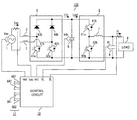•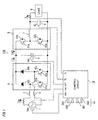•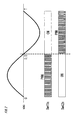•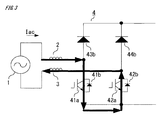•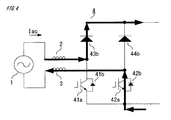•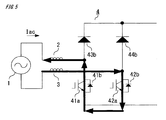•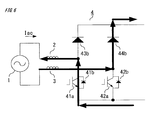•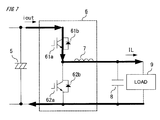•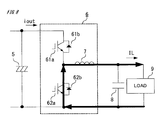•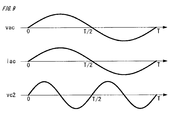•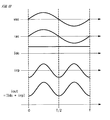•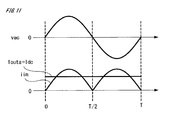•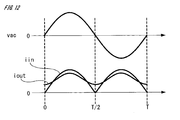•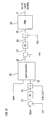•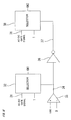•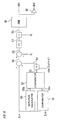•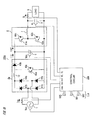•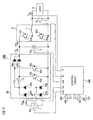•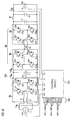•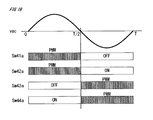•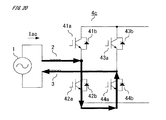•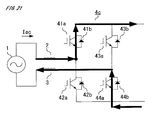•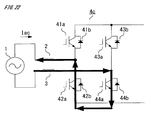•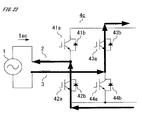•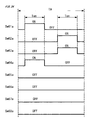•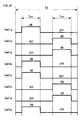•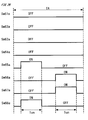•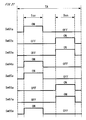•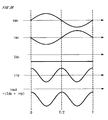•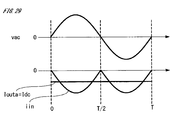•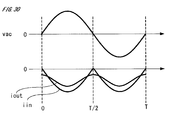•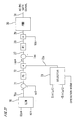•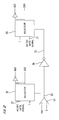•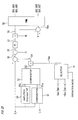•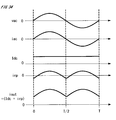•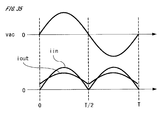•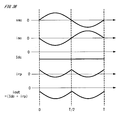•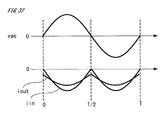•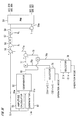•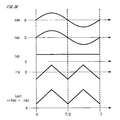•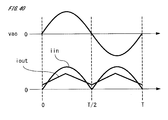•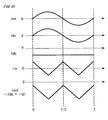•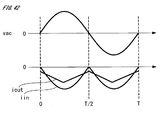•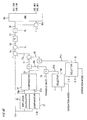•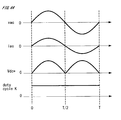•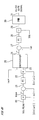•## Classifications

• HELECTRICITY
• H02GENERATION; CONVERSION OR DISTRIBUTION OF ELECTRIC POWER
• H02MAPPARATUS FOR CONVERSION BETWEEN AC AND AC, BETWEEN AC AND DC, OR BETWEEN DC AND DC, AND FOR USE WITH MAINS OR SIMILAR POWER SUPPLY SYSTEMS; CONVERSION OF DC OR AC INPUT POWER INTO SURGE OUTPUT POWER; CONTROL OR REGULATION THEREOF
• H02M1/00Details of apparatus for conversion
• H02M1/42Circuits or arrangements for compensating for or adjusting power factor in converters or inverters
• H02M1/4208Arrangements for improving power factor of AC input
• H02M1/4233Arrangements for improving power factor of AC input using a bridge converter consisting of active switches
• HELECTRICITY
• H02GENERATION; CONVERSION OR DISTRIBUTION OF ELECTRIC POWER
• H02MAPPARATUS FOR CONVERSION BETWEEN AC AND AC, BETWEEN AC AND DC, OR BETWEEN DC AND DC, AND FOR USE WITH MAINS OR SIMILAR POWER SUPPLY SYSTEMS; CONVERSION OF DC OR AC INPUT POWER INTO SURGE OUTPUT POWER; CONTROL OR REGULATION THEREOF
• H02M1/00Details of apparatus for conversion
• H02M1/08Circuits specially adapted for the generation of control voltages for semiconductor devices incorporated in static converters
• H02M1/083Circuits specially adapted for the generation of control voltages for semiconductor devices incorporated in static converters for the ignition at the zero crossing of the voltage or the current
• HELECTRICITY
• H02GENERATION; CONVERSION OR DISTRIBUTION OF ELECTRIC POWER
• H02MAPPARATUS FOR CONVERSION BETWEEN AC AND AC, BETWEEN AC AND DC, OR BETWEEN DC AND DC, AND FOR USE WITH MAINS OR SIMILAR POWER SUPPLY SYSTEMS; CONVERSION OF DC OR AC INPUT POWER INTO SURGE OUTPUT POWER; CONTROL OR REGULATION THEREOF
• H02M1/00Details of apparatus for conversion
• H02M1/14Arrangements for reducing ripples from dc input or output
• HELECTRICITY
• H02GENERATION; CONVERSION OR DISTRIBUTION OF ELECTRIC POWER
• H02MAPPARATUS FOR CONVERSION BETWEEN AC AND AC, BETWEEN AC AND DC, OR BETWEEN DC AND DC, AND FOR USE WITH MAINS OR SIMILAR POWER SUPPLY SYSTEMS; CONVERSION OF DC OR AC INPUT POWER INTO SURGE OUTPUT POWER; CONTROL OR REGULATION THEREOF
• H02M3/00Conversion of dc power input into dc power output
• H02M3/02Conversion of dc power input into dc power output without intermediate conversion into ac
• H02M3/04Conversion of dc power input into dc power output without intermediate conversion into ac by static converters
• H02M3/10Conversion of dc power input into dc power output without intermediate conversion into ac by static converters using discharge tubes with control electrode or semiconductor devices with control electrode
• H02M3/145Conversion of dc power input into dc power output without intermediate conversion into ac by static converters using discharge tubes with control electrode or semiconductor devices with control electrode using devices of a triode or transistor type requiring continuous application of a control signal
• H02M3/155Conversion of dc power input into dc power output without intermediate conversion into ac by static converters using discharge tubes with control electrode or semiconductor devices with control electrode using devices of a triode or transistor type requiring continuous application of a control signal using semiconductor devices only
• HELECTRICITY
• H02GENERATION; CONVERSION OR DISTRIBUTION OF ELECTRIC POWER
• H02MAPPARATUS FOR CONVERSION BETWEEN AC AND AC, BETWEEN AC AND DC, OR BETWEEN DC AND DC, AND FOR USE WITH MAINS OR SIMILAR POWER SUPPLY SYSTEMS; CONVERSION OF DC OR AC INPUT POWER INTO SURGE OUTPUT POWER; CONTROL OR REGULATION THEREOF
• H02M3/00Conversion of dc power input into dc power output
• H02M3/02Conversion of dc power input into dc power output without intermediate conversion into ac
• H02M3/04Conversion of dc power input into dc power output without intermediate conversion into ac by static converters
• H02M3/10Conversion of dc power input into dc power output without intermediate conversion into ac by static converters using discharge tubes with control electrode or semiconductor devices with control electrode
• H02M3/145Conversion of dc power input into dc power output without intermediate conversion into ac by static converters using discharge tubes with control electrode or semiconductor devices with control electrode using devices of a triode or transistor type requiring continuous application of a control signal
• H02M3/155Conversion of dc power input into dc power output without intermediate conversion into ac by static converters using discharge tubes with control electrode or semiconductor devices with control electrode using devices of a triode or transistor type requiring continuous application of a control signal using semiconductor devices only
• H02M3/156Conversion of dc power input into dc power output without intermediate conversion into ac by static converters using discharge tubes with control electrode or semiconductor devices with control electrode using devices of a triode or transistor type requiring continuous application of a control signal using semiconductor devices only with automatic control of output voltage or current, e.g. switching regulators
• H02M3/158Conversion of dc power input into dc power output without intermediate conversion into ac by static converters using discharge tubes with control electrode or semiconductor devices with control electrode using devices of a triode or transistor type requiring continuous application of a control signal using semiconductor devices only with automatic control of output voltage or current, e.g. switching regulators including plural semiconductor devices as final control devices for a single load
• HELECTRICITY
• H02GENERATION; CONVERSION OR DISTRIBUTION OF ELECTRIC POWER
• H02MAPPARATUS FOR CONVERSION BETWEEN AC AND AC, BETWEEN AC AND DC, OR BETWEEN DC AND DC, AND FOR USE WITH MAINS OR SIMILAR POWER SUPPLY SYSTEMS; CONVERSION OF DC OR AC INPUT POWER INTO SURGE OUTPUT POWER; CONTROL OR REGULATION THEREOF
• H02M3/00Conversion of dc power input into dc power output
• H02M3/22Conversion of dc power input into dc power output with intermediate conversion into ac
• H02M3/24Conversion of dc power input into dc power output with intermediate conversion into ac by static converters
• H02M3/28Conversion of dc power input into dc power output with intermediate conversion into ac by static converters using discharge tubes with control electrode or semiconductor devices with control electrode to produce the intermediate ac
• HELECTRICITY
• H02GENERATION; CONVERSION OR DISTRIBUTION OF ELECTRIC POWER
• H02MAPPARATUS FOR CONVERSION BETWEEN AC AND AC, BETWEEN AC AND DC, OR BETWEEN DC AND DC, AND FOR USE WITH MAINS OR SIMILAR POWER SUPPLY SYSTEMS; CONVERSION OF DC OR AC INPUT POWER INTO SURGE OUTPUT POWER; CONTROL OR REGULATION THEREOF
• H02M7/00Conversion of ac power input into dc power output; Conversion of dc power input into ac power output
• H02M7/02Conversion of ac power input into dc power output without possibility of reversal
• H02M7/04Conversion of ac power input into dc power output without possibility of reversal by static converters
• HELECTRICITY
• H02GENERATION; CONVERSION OR DISTRIBUTION OF ELECTRIC POWER
• H02MAPPARATUS FOR CONVERSION BETWEEN AC AND AC, BETWEEN AC AND DC, OR BETWEEN DC AND DC, AND FOR USE WITH MAINS OR SIMILAR POWER SUPPLY SYSTEMS; CONVERSION OF DC OR AC INPUT POWER INTO SURGE OUTPUT POWER; CONTROL OR REGULATION THEREOF
• H02M7/00Conversion of ac power input into dc power output; Conversion of dc power input into ac power output
• H02M7/02Conversion of ac power input into dc power output without possibility of reversal
• H02M7/04Conversion of ac power input into dc power output without possibility of reversal by static converters
• H02M7/12Conversion of ac power input into dc power output without possibility of reversal by static converters using discharge tubes with control electrode or semiconductor devices with control electrode
• HELECTRICITY
• H02GENERATION; CONVERSION OR DISTRIBUTION OF ELECTRIC POWER
• H02MAPPARATUS FOR CONVERSION BETWEEN AC AND AC, BETWEEN AC AND DC, OR BETWEEN DC AND DC, AND FOR USE WITH MAINS OR SIMILAR POWER SUPPLY SYSTEMS; CONVERSION OF DC OR AC INPUT POWER INTO SURGE OUTPUT POWER; CONTROL OR REGULATION THEREOF
• H02M7/00Conversion of ac power input into dc power output; Conversion of dc power input into ac power output
• H02M7/66Conversion of ac power input into dc power output; Conversion of dc power input into ac power output with possibility of reversal
• H02M7/68Conversion of ac power input into dc power output; Conversion of dc power input into ac power output with possibility of reversal by static converters
• H02M7/72Conversion of ac power input into dc power output; Conversion of dc power input into ac power output with possibility of reversal by static converters using discharge tubes with control electrode or semiconductor devices with control electrode
• H02M7/79Conversion of ac power input into dc power output; Conversion of dc power input into ac power output with possibility of reversal by static converters using discharge tubes with control electrode or semiconductor devices with control electrode using devices of a triode or transistor type requiring continuous application of a control signal
• H02M7/797Conversion of ac power input into dc power output; Conversion of dc power input into ac power output with possibility of reversal by static converters using discharge tubes with control electrode or semiconductor devices with control electrode using devices of a triode or transistor type requiring continuous application of a control signal using semiconductor devices only
• HELECTRICITY
• H02GENERATION; CONVERSION OR DISTRIBUTION OF ELECTRIC POWER
• H02MAPPARATUS FOR CONVERSION BETWEEN AC AND AC, BETWEEN AC AND DC, OR BETWEEN DC AND DC, AND FOR USE WITH MAINS OR SIMILAR POWER SUPPLY SYSTEMS; CONVERSION OF DC OR AC INPUT POWER INTO SURGE OUTPUT POWER; CONTROL OR REGULATION THEREOF
• H02M1/00Details of apparatus for conversion
• H02M2001/0003Details of control, feedback and regulation circuits
• H02M2001/0016Control circuits providing compensation of output voltage deviations using feedforward of disturbance parameter
• H02M2001/0022Control circuits providing compensation of output voltage deviations using feedforward of disturbance parameter the disturbance parameter being input voltage fluctuations
• YGENERAL TAGGING OF NEW TECHNOLOGICAL DEVELOPMENTS; GENERAL TAGGING OF CROSS-SECTIONAL TECHNOLOGIES SPANNING OVER SEVERAL SECTIONS OF THE IPC; TECHNICAL SUBJECTS COVERED BY FORMER USPC CROSS-REFERENCE ART COLLECTIONS [XRACs] AND DIGESTS
• Y02TECHNOLOGIES OR APPLICATIONS FOR MITIGATION OR ADAPTATION AGAINST CLIMATE CHANGE
• Y02BCLIMATE CHANGE MITIGATION TECHNOLOGIES RELATED TO BUILDINGS, e.g. HOUSING, HOUSE APPLIANCES OR RELATED END-USER APPLICATIONS
• Y02B70/00Technologies for an efficient end-user side electric power management and consumption
• Y02B70/10Technologies improving the efficiency by using switched-mode power supplies [SMPS], i.e. efficient power electronics conversion
• Y02B70/12Power factor correction technologies for power supplies
• Y02B70/126Active technologies

## Abstract

A main circuit of a power conversion device includes: an AC/DC converter for performing power factor correction control for a single-phase AC power supply; and a DC/DC converter connected to the AC/DC converter via a DC capacitor. In order to reduce ripple voltage and ripple current for the DC capacitor, a control circuit superimposes, onto a DC current command, an AC current command having the minimum value at the zero cross phase of the single-phase AC power supply and having the maximum value at the peak phase thereof, to generate an output current command for the DC/DC converter, and performs output control for the DC/DC converter, using the output current command.

## Description

TECHNICAL FIELD

The present invention relates to a power conversion device in which an AC/DC converter for converting power while performing power factor correction control for an AC power supply, and a DC/DC converter are connected to each other via a DC capacitor.

BACKGROUND ART

In a motor control device as a conventional power conversion device, an AC power supply is rectified by a rectification circuit unit, and a high-power-factor converter circuit unit steps up inputted DC voltage and outputs the resultant voltage. The high-power-factor converter circuit unit controls the time ratio of ON and OFF of a switching transistor in a sinewave manner, thereby improving the power factor, and controls the DC voltage. The stepped-up DC voltage is stabilized by a smoothing capacitor, and an inverter circuit unit converts the DC voltage supplied from the smoothing capacitor, to three-phase AC, and supplies the resultant voltage to a motor, to drive the motor. In the case where the smoothing capacitor has a small capacitance, if, in particular, the commercial AC power supply is a single-phase type, ripple voltage of DC bus voltage, which varies at a frequency twice as high as the power supply frequency, increases.

The voltage applied to the motor varies due to the ripple voltage of the DC bus voltage, whereby the motor phase current pulsates. In order to remove the pulsation, a control unit outputs, to the inverter circuit unit, a voltage pulse corrected so as not to be influenced by the DC bus ripple voltage, by applying, to DC bus voltage, a feedback value of DC bus voltage detected by DC bus voltage detection means when calculating a current command, and thus drives the motor (see, for example, Patent Document 1).

CITATION LIST Patent Document

Patent Document 1: Japanese Laid-Open Patent Publication No. 2002-291260

SUMMARY OF THE INVENTION Problems to be Solved by the Invention

In such a conventional power conversion device, since the inverter circuit unit is controlled so that power pulsation having a frequency twice as high as the frequency of the power supply voltage does not flow out to a load, voltage ripple at the smoothing capacitor increases. If the voltage ripple is great, the maximum voltage causes overvoltage in the power conversion device, or due to the minimum voltage, output voltage from the inverter circuit unit cannot be ensured, and thus it is difficult to reliably operate the power conversion device. In addition, there is a problem that increasing the capacitance of the capacitor in order to reduce the voltage ripple leads to increase in the size of the power conversion device.

The present invention has been made to solve the above problems, and an object of the present invention is to obtain a power conversion device that reduces voltage oscillation of a DC capacitor by outputting permissible power pulsation, and that has a small size and high reliability.

Solution to the Problems

A first power conversion device according to the present invention includes: an AC/DC converter for performing power factor correction control for a single-phase AC power supply and converting AC power from the single-phase AC power supply, to DC power; a DC/DC converter which is connected to a DC side of the AC/DC converter and performs voltage conversion of DC power; a DC capacitor connected between positive and negative DC buses between the AC/DC converter and the DC/DC converter; and a control circuit for performing output control for the AC/DC converter and the DC/DC converter. The control circuit superimposes, onto a DC current command, an AC current command having a minimum value at a zero cross phase of the single-phase AC power supply and having a maximum value at a peak phase thereof, to generate an output current command for the DC/DC converter, and performs output control for the DC/DC converter, using the output current command.

A second power conversion device according to the present invention includes: an AC/DC converter for performing power factor correction control for a single-phase AC power supply and converting AC power from the single-phase AC power supply, to DC power; a DC/DC converter which is connected to a DC side of the AC/DC converter and performs voltage conversion of DC power; a DC capacitor connected between positive and negative DC buses between the AC/DC converter and the DC/DC converter; and a control circuit for performing output control for the AC/DC converter and the DC/DC converter. The control circuit controls the DC/DC converter with a constant duty cycle, generates a voltage command for the DC capacitor so as to contain an AC voltage component, and performs output control for the AC/DC converter, using an output current command based on the voltage command.

Effect of the Invention

The first and second power conversion devices of the present invention cause the DC/DC converter to output permissible power pulsation and thereby can reduce voltage oscillation of the DC capacitor without increasing the capacitance of the DC capacitor. Thus, it becomes possible to improve the reliability of the power conversion devices and promote downsizing thereof.

BRIEF DESCRIPTION OF THE DRAWINGS

FIG. 1 is a configuration diagram of a power conversion device according to embodiment 1 of the present invention.

FIG. 2 is a diagram illustrating operation of an AC/DC converter according to embodiment 1 of the present invention.

FIG. 3 is a current route diagram illustrating operation of the AC/DC converter according to embodiment 1 of the present invention.

FIG. 4 is a current route diagram illustrating operation of the AC/DC converter according to embodiment 1 of the present invention.

FIG. 5 is a current route diagram illustrating operation of the AC/DC converter according to embodiment 1 of the present invention.

FIG. 6 is a current route diagram illustrating operation of the AC/DC converter according to embodiment 1 of the present invention.

FIG. 7 is a current route diagram illustrating operation of a DC/DC converter according to embodiment 1 of the present invention.

FIG. 8 is a current route diagram illustrating operation of the DC/DC converter according to embodiment 1 of the present invention.

FIG. 9 is a waveform diagram showing voltage and current of an AC power supply and ripple voltage of a DC capacitor in a reference example of embodiment 1 of the present invention.

FIG. 10 is a waveform diagram showing each component contained in output current of the DC capacitor according to embodiment 1 of the present invention.

FIG. 11 is a waveform diagram showing input and output currents of the DC capacitor in a comparative example of embodiment 1 of the present invention.

FIG. 12 is a waveform diagram showing input and output currents of the DC capacitor according to embodiment 1 of the present invention.

FIG. 13 is a control block diagram showing generation of a gate signal for the AC/DC converter according to embodiment 1 of the present invention.

FIG. 14 is a control block diagram showing generation of gate signals for the AC/DC converter according to embodiment 1 of the present invention.

FIG. 15 is a control block diagram showing generation of gate signals for the DC/DC converter according to embodiment 1 of the present invention.

FIG. 16 is a configuration diagram of a power conversion device in another example of embodiment 1 of the present invention.

FIG. 17 is a configuration diagram of a power conversion device in still another example of embodiment 1 of the present invention.

FIG. 18 is a configuration diagram of a power conversion device according to embodiment 2 of the present invention.

FIG. 19 is a diagram illustrating operation of an AC/DC converter according to embodiment 2 of the present invention.

FIG. 20 is a current route diagram illustrating operation of the AC/DC converter according to embodiment 2 of the present invention.

FIG. 21 is a current route diagram illustrating operation of the AC/DC converter according to embodiment 2 of the present invention.

FIG. 22 is a current route diagram illustrating operation of the AC/DC converter according to embodiment 2 of the present invention.

FIG. 23 is a current route diagram illustrating operation of the AC/DC converter according to embodiment 2 of the present invention.

FIG. 24 is a waveform diagram illustrating power-running operation of a DC/DC converter according to embodiment 2 of the present invention.

FIG. 25 is a waveform diagram illustrating another example of power-running operation of the DC/DC converter according to embodiment 2 of the present invention.

FIG. 26 is a waveform diagram illustrating regeneration operation of the DC/DC converter according to embodiment 2 of the present invention.

FIG. 27 is a waveform diagram illustrating still another example of regeneration operation of the DC/DC converter according to embodiment 2 of the present invention.

FIG. 28 is a waveform diagram showing each component of output current of a DC capacitor in regeneration operation of the power conversion device according to embodiment 2 of the present invention.

FIG. 29 is a waveform diagram showing input and output currents of the DC capacitor in regeneration operation of the power conversion device in a comparative example of embodiment 2 of the present invention.

FIG. 30 is a waveform diagram showing input and output currents of the DC capacitor in regeneration operation of the power conversion device according to embodiment 2 of the present invention.

FIG. 31 is a control block diagram showing generation of a gate signal for the AC/DC converter according to embodiment 2 of the present invention.

FIG. 32 is a control block diagram showing generation of gate signals for the AC/DC converter according to embodiment 2 of the present invention.

FIG. 33 is a control block diagram showing generation of gate signals for the DC/DC converter according to embodiment 2 of the present invention.

FIG. 34 is a waveform diagram showing each component of output current of a DC capacitor in power-running operation of a power conversion device according to embodiment 3 of the present invention.

FIG. 35 is a waveform diagram showing input and output currents of the DC capacitor in power-running operation of the power conversion device according to embodiment 3 of the present invention.

FIG. 36 is a waveform diagram showing each component of output current in the DC capacitor in regeneration operation of the power conversion device according to embodiment 3 of the present invention.

FIG. 37 is a waveform diagram showing input and output currents of the DC capacitor in regeneration operation of the power conversion device according to embodiment 3 of the present invention.

FIG. 38 is a control block diagram showing generation of gate signals for a DC/DC converter according to embodiment 3 of the present invention.

FIG. 39 is a waveform diagram showing each component of output current of a DC capacitor in power-running operation of a power conversion device according to embodiment 4 of the present invention.

FIG. 40 is a waveform diagram showing input and output currents of the DC capacitor in power-running operation of the power conversion device according to embodiment 4 of the present invention.

FIG. 41 is a waveform diagram showing each component of output current of the DC capacitor in regeneration operation of the power conversion device according to embodiment 4 of the present invention.

FIG. 42 is a waveform diagram showing input and output currents of the DC capacitor in regeneration operation of the power conversion device according to embodiment 4 of the present invention.

FIG. 43 is a control block diagram showing generation of gate signals for a DC/DC converter according to embodiment 4 of the present invention.

FIG. 44 is a waveform diagram at each section, illustrating operation of a power conversion device according to embodiment 5 of the present invention.

FIG. 45 is a control block diagram showing generation of a gate signal for an AC/DC converter according to embodiment 5 of the present invention.

FIG. 46 is a control block diagram showing generation of gate signals for a DC/DC converter according to embodiment 5 of the present invention.

DESCRIPTION OF EMBODIMENTS Embodiment 1

Hereinafter, a power conversion device according to embodiment 1 of the present invention will be described. FIG. 1 is a schematic configuration diagram of the power conversion device according to embodiment 1 of the present invention.

As shown in FIG. 1, the power conversion device 100 includes: a main circuit for converting AC power from a single-phase AC power supply 1 (hereinafter, simply referred to as an AC power supply 1), to DC power, and outputting the DC power to a load 9; and a control circuit 10.

The main circuit includes: an AC/DC converter 4 for performing power factor correction control for the AC power supply 1 and converting AC power to DC power; a DC/DC converter 6 which is connected to the DC side of the AC/DC converter 4 and performs voltage conversion of the DC power; and a DC capacitor 5 connected between DC buses between the AC/DC converter 4 and the DC/DC converter 6. In addition, reactors 2 and 3 for current limitation are provided on the input side, and a smoothing capacitor 8 is provided on the output side.

In this case, the AC/DC converter 4 is configured as a semi-bridgeless circuit, and includes semiconductor switching elements 41 a, 42 a and diode elements 43 b, 44 b. The semiconductor switching elements 41 a, 42 a are formed from IGBTs (Insulated Gate Bipolar Transistors), to which diodes 41 b, 42 b are respectively connected in antiparallel.

In this case, the DC/DC converter 6 is configured as a step-down chopper circuit of a non-isolation type, and includes semiconductor switching elements 61 a, 62 a, and a reactor 7 for current control. The semiconductor switching elements 61 a, 62 a are formed from IGBTs, to which diodes 61 b, 62 b are respectively connected in antiparallel.

It is noted that, instead of IGBTs, the semiconductor switching elements 41 a, 42 a, 61 a, 62 a may be MOSFETs (Metal Oxide Semiconductor Field Effect Transistors) including diodes between the sources and drains, or may be semiconductor switching elements such as thyristors. In the case of using MOSFETs, the included diodes may be used as the diodes 41 b, 42 b, 61 b, 62 b.

A P-side terminal of the DC capacitor 5 is connected to a P terminal of the DC output side of the AC/DC converter 4, and a P terminal on the DC input side of the DC/DC converter 6. An N-side terminal of the DC capacitor 5 is connected to an N terminal on the DC output side of the AC/DC converter 4, and an N terminal on the DC input side of the DC/DC converter 6.

The DC capacitor 5 has an energy buffer function, and smooths the difference between power inputted from the AC/DC converter 4 and power outputted from the DC/DC converter 6. The DC capacitor 5 may be formed from an aluminum electrolytic capacitor, a film capacitor, a ceramic capacitor, a tantalum capacitor, an EDLC (electric double layered capacitor), or the like. Alternatively, the DC capacitor 5 may be formed by a battery such as a lithium ion battery.

The load 9 may be an electric apparatus driven with DC voltage, or a power storage element such as a battery or a capacitor.

AC voltage vac of the AC power supply 1, voltage vc1 of the DC capacitor 5, and load voltage VL which is voltage of the smoothing capacitor 8, are detected by voltage sensors (not shown), and the detected voltages are inputted to the control circuit 10. Further, AC current iac of the AC power supply 1 and load current IL are detected by current sensors, and the detected currents are inputted to the control circuit 10. The control circuit 10 generates gate signals 11 (gate signals G41, G42, G61, G62 for the semiconductor switching elements 41 a, 42 a, 61 a, 62 a) on the basis of the inputted voltage current information, to perform output control for the AC/DC converter 4 and the DC/DC converter 6.

It is noted that Vac indicates a voltage effective value of the AC power supply 1, Iac indicates a current effective value of the AC power supply 1, Vdc indicates a DC voltage component of the DC capacitor 5, iin indicates input current of the DC capacitor 5, and iout indicates output current of the DC capacitor 5.

The operation of the power conversion device 100 configured as described above will be described below.

FIG. 2 is a diagram illustrating operation of the AC/DC converter 4. In FIG. 2, Sw41 a and Sw42 a indicate the switching states of the semiconductor switching elements 41 a and 42 a.

One cycle of the AC power supply 1 is denoted by T. During the period from time 0 to time T/2, voltage vac of the AC power supply 1 has a positive polarity, and the control circuit 10 turns on the semiconductor switching element 42 a and performs PWM control for the semiconductor switching element 41 a, to perform control so that the input power factor from the AC power supply 1 becomes substantially 1, that is, to control current iac at a high power factor. During the period from time T/2 to time T, voltage vac of the AC power supply 1 has a negative polarity, and the control circuit 10 turns on the semiconductor switching element 41 a and performs PWM control for the semiconductor switching element 42 a, to perform control so that the input power factor from the AC power supply 1 becomes substantially 1, that is, to control current iac at a high power factor.

FIG. 3 to FIG. 6 are current route diagrams illustrating operation of the AC/DC converter 4.

During the period from time 0 to time T/2, the semiconductor switching element 42 a is kept in an ON state, and when the semiconductor switching element 41 a is turned on, the input current is short-circuited via the reactors 2 and 3, and the reactor 2 and the reactor 3 are excited, so that the current increases in the positive direction (FIG. 3). Then, when the semiconductor switching element 41 a is turned off, excitation energy accumulated in the reactor 2 and the reactor 3 is outputted to the DC capacitor 5 side via the diode 43 b. At this time, the current in the reactors 2 and 3 decreases (FIG. 4).

During the period from time T/2 to time T, the semiconductor switching element 41 a is kept in an ON state, and when the semiconductor switching element 42 a is turned on, the input current is short-circuited via the reactors 2 and 3, and the reactor 2 and the reactor 3 are excited, so that the current increases in the negative direction (FIG. 5). Then, when the semiconductor switching element 42 a is turned off, excitation energy accumulated in the reactor 2 and the reactor 3 is outputted to the DC capacitor 5 side via the diode 44 b. At this time, the current in the reactors 2 and 3 decreases (FIG. 6).

The control circuit 10 performs high power factor control for the inputted AC current iac by performing ON/OFF control for the semiconductor switching elements 41 a, 42 a as described above. It is noted that ideally, the semiconductor switching element 41 a and the semiconductor switching element 42 a are driven with the same duty cycle.

Here, a theoretical duty cycle D41 for the semiconductor switching element 41 a when the current iac is controlled at a high power factor is shown in the following expression (1). In this case, a duty cycle D43 for the diode 43 b is represented by expression (2) on the basis of expression (1). It is noted that voltage vac of the AC power supply 1 is defined by expression (3). Therefore, current iin flowing into the DC capacitor 5 can be calculated by expression (4).

It is noted that it is assumed that there is no loss between the AC power supply 1 and the DC capacitor 5.
D41=(Vdc−vac)/Vdc  (1)
D43=vac/Vdc  (2)
vac=(√{square root over ( )}2)Vac·sin ωt  (3)
iin=(vac/Vdc)iac=(2Vac·Iac/Vdc)sin2 ωt  (4)

Next, operation of the DC/DC converter 6 will be described.

A step-down chopper circuit is used for the DC/DC converter 6. The control circuit 10 performs ON/OFF control for the semiconductor switching elements 61 a, 62 a, thereby causing the DC capacitor 5 to output DC power and controlling current IL and voltage VL to the load 9, to be desired values.

FIG. 7 and FIG. 8 are current route diagrams illustrating operation of the DC/DC converter 6.

During a period in which the semiconductor switching element 61 a is turned on, the semiconductor switching element 62 a is turned off, and current flows from the DC capacitor 5 to the semiconductor switching element 61 a, the reactor 7, and the load 9 (FIG. 7). During a period in which the semiconductor switching element 62 a is turned on, the semiconductor switching element 61 a is turned off. When the semiconductor switching element 62 a is turned on and the semiconductor switching element 61 a is turned off from the state in FIG. 7, current circulates through the semiconductor switching element 62 a and the reactor 7 to the load 9, due to the current continuity of the reactor 7 (FIG. 8).

For the DC/DC converter 6 which operates as described above, the control circuit 10 adjusts the duty cycle of the semiconductor switching element 61 a and the semiconductor switching element 62 a, thereby adjusting power supplied to the load 9, in this case, adjusting the load current IL. The DC/DC converter 6 supplies the current IL to the load 9 at the DC voltage VL, thereby supplying DC power to the load 9.

The current iout outputted from the DC capacitor 5 is discontinuous with respect to the switching cycles of the semiconductor switching elements 61 a, 62 a, but can be considered to be current that is continuous, on average, with respect to the cycle T of the AC power supply 1. Output current iout of the DC capacitor 5 is assumed to be DC current Idc. In this case, the voltage relational expression of the DC capacitor 5 can be represented by the following expression (5). Here, Cdc is the electrostatic capacitance of the DC capacitor 5, and vc2 is an AC voltage component (ripple voltage) of the DC capacitor 5. Under the assumption that the inputted AC current iac is subjected to high power factor control, the inputted AC current iac is represented by expression (6). By solving expression (5) for AC voltage component vc2 of the DC capacitor 5, expression (7) is derived.
Cdc(dvc2/dt)=iin−iout=(2Vac·Iac/Vdc)sin2 ωt−Idc  (5)
iac=(√{square root over ( )}2)Iac·sin ωt  (6)
vc2=(2Vac·Iac/2ωCdc·Vdc)sin(2ωt)  (7)

Expression (7) indicates that, if output current iout of the DC capacitor 5 is DC current, the ripple voltage vc2 that has a frequency twice as high as the frequency of the AC power supply 1 necessarily occurs on the DC capacitor 5, by the high power factor control performed in the AC/DC converter 4 connected to the AC power supply 1. FIG. 9 shows a waveform diagram of such ripple voltage vc2, and the voltage vac and current iac of the AC power supply 1, as a reference example of the present embodiment. As shown in FIG. 9, the ripple voltage vc2 greatly varies at a frequency twice as high as the frequency of the AC power supply 1.

In the present embodiment, in order to suppress the ripple voltage vc2 occurring on the DC capacitor 5, the control circuit 10 intentionally superimposes an AC current component (ripple current) irp onto output current iout of the DC capacitor 5. Specifically, the DC/DC converter 6 is controlled so that an AC current component (ripple current) is generated in current IL outputted to the load 9, thereby generating ripple current irp in output current iout of the DC capacitor 5.

FIG. 10 is a waveform diagram showing voltage vac and current iac of the AC power supply 1 and components contained in output current iout of the DC capacitor 5. The output current iout of the DC capacitor 5 is current obtained by superimposing ripple current irp onto the DC current component Idc, and the ripple current irp to be superimposed is sinewave current having a frequency twice as high as the frequency of voltage vac of the AC power supply 1. The initial phase of the ripple current irp is set such that the ripple current irp has the minimum value at the zero cross phase of the AC power supply 1 and has the maximum value at the peak phase thereof.

If voltage vac of the AC power supply 1 shown in FIG. 10 is defined as shown by the above expression (3) and AC current iac controlled at a power factor of 1 is defined as shown by the above expression (6), ripple current irp of the DC capacitor 5 is represented by the following expression (8), and output current iout is represented by the following expression (9). Here, Irp is the effective value of ripple current irp.
irp=−(√{square root over ( )}2)Irp·cos(2ωt)  (8)
iout=Idc−(√{square root over (0)}2)Irp·cos(2ωt)  (9)

FIG. 11 is a waveform diagram showing input and output currents of the DC capacitor 5 in the case where the DC capacitor 5 outputs only DC current, as a comparative example. In this case, output current iouta of the DC capacitor 5 becomes the DC current Idc.

As shown in FIG. 11, at the zero cross phase of the AC power supply 1, since input current iin to the DC capacitor 5 is almost zero, charge/discharge current (iin−iouta) compensated by the DC capacitor 5 becomes almost (−Idc), that is, the DC capacitor 5 mostly bears the outputted DC current Idc. On the other hand, at the peak phase, since input current iin has the maximum value, the charge/discharge current (iin−iouta) compensated by the DC capacitor 5 becomes surplus for charging, so that the surplus current flows into the DC capacitor 5 and charges the DC capacitor 5.

In the present embodiment, output current iout of the DC capacitor 5 includes the ripple current irp superimposed thereon as described above. FIG. 12 is a waveform diagram showing input and output currents of the DC capacitor 5.

As shown in FIG. 12, at the zero cross phase of the AC power supply 1, output current iout becomes the minimum value along with input current iin of the DC capacitor 5 becoming almost zero. At the peak phase, output current iout becomes the maximum value along with input current iin becoming the maximum value. Thus, the charge/discharge current (iin−iout), which is ripple current, compensated by the DC capacitor 5 can be suppressed, and the ripple voltage vc2 and the ripple current effective value of the DC capacitor 5 can be suppressed.

Since the DC capacitor 5 outputs the output current iout shown by the above expression (9), the voltage relational expression of the DC capacitor 5 can be represented by the following expression (10). By solving expression (10) for ripple voltage vc2 of the DC capacitor 5, expression (11) is derived.

$Cdc ⁡ ( dvc ⁢ ⁢ 2 / dt ) = ⁢ iin - iout = ⁢ ( 2 ⁢ Vac · Iac / Vdc ) ⁢ sin 2 ⁢ ω ⁢ ⁢ t - ⁢ ( Idc - ( 2 ) ⁢ Irp · cos ⁡ ( 2 ⁢ ω ⁢ ⁢ t ) ) ( 10 ) vc ⁢ ⁢ 2 = ( ( Vac · Iac - Vdc · ( 2 ) ⁢ Irp ) / 2 ⁢ ω ⁢ ⁢ Cdc · Vdc ) · sin ⁡ ( 2 ⁢ ω ⁢ ⁢ t ) ( 11 )$

As shown in the above expression (11), when the peak value (√{square root over ( )}2)Irp of ripple current irp superimposed on output current iout of the DC capacitor 5 increases, ripple voltage vc2 occurring on the DC capacitor 5 decreases.

An amplitude ΔVdc of ripple voltage vc2 which is an AC voltage component of the DC capacitor 5 is defined by the following expression (12) on the basis of the above expression (11).
ΔVdc=((Vac·Iac−Vdc·(√{square root over ( )}2)Irp)/2ωCdc·Vdc)  (12)

Input current iin flowing into the DC capacitor 5 can be represented by the following expression (13). Since output current iout flowing out from the DC capacitor 5 is represented by the above expression (9), charge/discharge current (iin−iout) of the DC capacitor 5 is represented by the following expression (14).

Expression (13) and expression (14) can be represented by expression (13a) and expression (14a) using load voltage VL and load current IL. Here, IL* is a command value for load current IL, and ILrp is the effective value of the ripple current to be generated in the load current IL.

$iin = ⁢ ( Vac · Iac / Vdc ) · ( 1 + cos ⁡ ( 2 ⁢ ω ⁢ ⁢ t - π ) ) = ⁢ ( VL · IL / Vdc ) · ( 1 + cos ⁡ ( 2 ⁢ ω ⁢ ⁢ t - π ) ) ( 13 ) ( 13 ⁢ a ) iin - iout = ⁢ ( Idc - ( 2 ) ⁢ Irp ) · cos ⁡ ( 2 ⁢ ω ⁢ ⁢ t - π ) = ⁢ ( VL / Vdc ) · ( IL * - ( 2 ) ⁢ ILrp ) · cos ⁡ ( 2 ⁢ ω ⁢ ⁢ t - π ) ( 14 ) ( 14 ⁢ a )$

As shown by the above expression (14a), it is found that charge/discharge current (iin−iout) of the DC capacitor 5 becomes a component having a frequency twice as high as the frequency of voltage vac of the AC power supply 1. In addition, the charge/discharge current (iin−iout) decreases when the peak value (√{square root over ( )}2)Irp of ripple current irp superimposed on output current iout increases, or the ripple current peak value (√{square root over ( )}2)ILrp generated in load current IL increases.

An amplitude ΔIrp of charge/discharge current (iin−iout) of the DC capacitor 5 is defined by the following expression (15) on the basis of the above expression (14a).
ΔIrp=(VL/Vdc)·(IL*−(√{square root over ( )}2)ILrp)  (15)

By the way, a current component having a carrier frequency of the AC/DC converter 4 and the DC/DC converter 6 flows into or from the DC capacitor 5. The charge/discharge current of the DC capacitor 5 is actually defined by the total sum including other frequency components such as the carrier frequency component, as well as the one shown by the above expression (14a). In particular, the carrier frequency is dominant and is greatly higher than the power supply frequency of the AC power supply 1, and the current component having the carrier frequency, which flows into or from the DC capacitor 5, is constant without depending on the frequency component twice as high as the frequency of the AC power supply 1. That is, of the charge/discharge current of the DC capacitor 5, the current component shown by expression (14a) varies but the current component having the carrier frequency is constant. Therefore, in the present embodiment, the current component shown by expression (14a) is suppressed, and the ripple current converges to the current component having the carrier frequency.

As described above, by the DC capacitor 5 outputting ripple current irp shown by the above expression (8), ripple voltage vc2 occurring on the DC capacitor 5 can be suppressed on the basis of the above expression (11), and charge/discharge current (iin−iout) compensated by the DC capacitor 5 can be suppressed on the basis of the above expression (14a). The ripple current irp outputted from the DC capacitor 5 is sinewave current having a frequency twice as high as the frequency of voltage vac of the AC power supply 1, and the initial phase of the ripple current irp is set such that the ripple current irp has the minimum value at the zero cross phase and has the maximum value at the peak phase. The DC/DC converter 6 causes the DC capacitor 5 to output such ripple current irp.

Next, control for the AC/DC converter 4 and the DC/DC converter 6 by the control circuit 10 will be described.

FIG. 13 is a control block diagram showing generation of a gate signal for the AC/DC converter 4, in the control circuit 10. In control for the AC/DC converter 4, the control circuit 10 controls current iac inputted from the AC power supply 1, at a power factor of 1 with respect to voltage vac of the AC power supply 1. Further, the control circuit 10 selectively performs voltage control for the DC capacitor 5.

In the case where the control circuit 10 performs constant voltage control for controlling voltage vc1 of the DC capacitor 5 to be constant, a deviation 21 between a DC voltage command value Vdc* and detected voltage vc1 is subjected to PI control, to generate a current command amplitude 22. Then, the current command amplitude 22 is multiplied by a sinewave signal sin ωt having the same phase as voltage vac of the AC power supply 1, to calculate a current command 23.

On the other hand, in control for the AC/DC converter 4, in the case where the control circuit 10 performs only high power factor control for current iac without performing constant voltage control for voltage vc1 of the DC capacitor 5, a current command iac* is prepared.

A switchover device 25 selects one of the current command 23 and the current command iac* as a current command 26, in accordance with whether or not to perform constant voltage control for the DC capacitor 5. Next, a deviation 27 between the current command 26 and detected current iac is subjected to PI control, to calculate a voltage command value 28, and the voltage command value 28 is divided by the DC voltage component Vdc of the DC capacitor 5, thereby calculating a duty cycle 29. Then, a PWM circuit 30 generates a gate signal 31 for performing PWM control for the AC/DC converter 4, on the basis of the duty cycle 29. In the PWM circuit 30, the carrier frequency can be arbitrarily adjusted, and a triangle wave, a saw-tooth wave, or the like is used as the carrier wave.

FIG. 14 is a control block diagram showing generation of gate signals G41, G42 for the respective semiconductor switching elements 41 a, 42 a in the AC/DC converter 4, in the control circuit 10.

The gate signal 31 is inputted to a selector 32 for the semiconductor switching element 41 a, and a selector 38 for the semiconductor switching element 42 a. A polarity determination device 33 determines the polarity of voltage vac of the AC power supply 1, and outputs a signal 34 which indicates 1 when the voltage vac is positive, and indicates 0 when the voltage vac is negative.

On the basis of the signal 34 from the polarity determination device 33, the selector 32 selects the gate signal 31 when the voltage vac is positive, and selects 1, i.e., an ON signal, when the voltage vac is negative, thus obtaining the gate signal G41. Meanwhile, on the basis of a signal 37 obtained by an inversion device 36 inverting the signal 34, the selector 38 selects the gate signal 31, or 1. That is, the selector 38 selects the gate signal 31 when the voltage vac is negative, and selects 1, i.e., an ON signal, when the voltage vac is positive, thus obtaining the gate signal G42.

Thus, in accordance with the control shown in FIG. 13, the control circuit 10 generates the duty cycle 29 to perform high power factor control for current iac, and as necessary, perform constant voltage control for the DC capacitor 5, and thereby generates the gate signal 31 for the AC/DC converter 4. Then, in accordance with the control shown in FIG. 14, the control circuit 10 selects one of the semiconductor switching elements 41 a, 42 a in response to the polarity of voltage vac of the AC power supply 1, to apply the gate signal 31 to the selected element and control the same, and turns on the other element.

FIG. 15 is a control block diagram showing generation of gate signals G61, G62 for the respective semiconductor switching elements 61 a, 62 a in the DC/DC converter 6, in the control circuit 10.

A command value IL* for load current IL outputted to the load 9 is a DC current command having only a DC component, and FIG. 15 shows constant current control for supplying constant DC current IL to the load 9.

As shown in FIG. 15, an amplitude calculator 50 calculates a ripple current peak value 50 a on the basis of the command value IL*.

The above expression (11) is a reduction theoretical expression for ripple voltage vc2 of the DC capacitor 5, and the ripple current peak value 50 a is calculated using the expression (11). By deforming the above expression (12) obtained from expression (11), the ripple current peak value (√{square root over ( )}2)Irp can be calculated on the basis of the amplitude ΔVdc of ripple voltage vc2 of the DC capacitor 5. A command value ((√{square root over ( )}2)Irp)* for this (√{square root over ( )}2) Irp can be calculated from the following expression (16) using an amplitude target value ΔVdc* for ripple voltage vc2, and the ((√{square root over ( )}2)Irp)* is used as the ripple current peak value 50 a. In this case, theoretically, the ripple current peak value 50 a is a target peak value for ripple current irp to be superimposed onto output current iout of the DC capacitor 5.

$( ( 2 ) ⁢ Irp ) * = ⁢ ( Vac · Iac - 2 ⁢ ω ⁢ ⁢ Cdc · Vdc · Δ ⁢ ⁢ Vdc * ) / Vdc = ⁢ ( VL / Vdc ) ⁢ IL * - 2 ⁢ ω ⁢ ⁢ Cdc · Δ ⁢ ⁢ Vdc * ( 16 )$

It is noted that the ripple current peak value 50 a may be calculated using the above expression (14a) which is a reduction theoretical expression for charge/discharge current of the DC capacitor 5. In this case, by deforming expression (15) which is based on expression (14a), the peak value (√{square root over ( )}2)ILrp of the ripple current to be generated in the load current IL can be calculated on the basis of the amplitude ΔIrp of charge/discharge current of the DC capacitor 5. A command value ((√{square root over ( )}2)ILrp)* for this (√{square root over ( )}2)ILrp can be calculated from the following expression (17) using an amplitude target value ΔIrp* for charge/discharge current of the DC capacitor 5, and the ((√{square root over ( )}2)ILrp)* is used as the ripple current peak value 50 a. In this case, theoretically, the ripple current peak value 50 a is a target peak value for the ripple current to be generated in the load current IL.
((√{square root over ( )}2)ILrp)*=IL*−(Vdc/VL)·ΔIrp*  (17)

A limitation value 51 a is provided for the ripple current peak value 50 a. A comparator 51 compares the command value IL* for load current IL and a limitation value Lim set in advance for the load 9, and outputs the lower value as the limitation value 51 a. The reason for limitation by the command value IL* is to prevent the instantaneous value of current supplied to the load 9 from becoming smaller than 0 A, so as not to cause a discontinuous mode. The limitation value Lim set for the load 9 is, for example, in the case where the load 9 is assumed to be a battery, a value prescribed from lifetime deterioration due to heat generation of the battery caused by increase in the AC component.

A comparator 52 compares the ripple current peak value 50 a and the limitation value 51 a, and the comparator 52 outputs the lower value as an amplitude 53 of an AC current command. The control circuit 10 multiplies the amplitude 53 by a function P shown by the following expression (18) using w as the angular frequency of AC voltage vac shown by the above expression (3), thereby calculating a ripple current command 54 as the AC current command.
P=cos(2ωt−π)  (18)

It is noted that the function P by which the amplitude 53 of the AC current command is multiplied may be a function representing a sinewave having the minimum value at the zero cross phase of AC voltage vac and having the maximum value at the peak phase thereof. That is, the function P can be represented by the following expression (19) using an angular frequency (2(2n−1)ω). It is noted that n is a natural number, and in the case of n=1, the angular frequency is 2ω and the function P becomes the above expression (18).

That is, the ripple current command 54 generated by multiplying the amplitude 53 by the function P is generated with the phase thereof lagging by (π/2) relative to a fundamental sinewave having a frequency 2×(2n−1) times as high as the fundamental frequency of the AC power supply 1.
P=cos(2(2n−1)ωt−π)  (19)

The control circuit 10 adds the calculated ripple current command 54 to the command value IL* for load current IL, thereby generating a current command value 55 containing ripple current, as an output current command for the DC/DC converter 6.

Next, the current command value 55 is compared with detected load current IL, and the resultant deviation 56 is subjected to PI control, to calculate a voltage command value 57. The voltage command value 57 is divided by DC voltage VL of the load 9, to calculate a duty cycle 58. Then, on the basis of the duty cycle 58 and using a carrier signal, a PWM circuit 59 generates a gate signal G61 for the semiconductor switching element 61 a in the DC/DC converter 6. An inversion device 60 inverts the gate signal G61, to generate a gate signal G62 for the semiconductor switching element 62 a.

Although it is not necessary to provide a dead time for the semiconductor switching elements 41 a, 42 a in the AC/DC converter 4, a dead time may be provided for the semiconductor switching elements 61 a, 62 a in the DC/DC converter 6.

In the present embodiment, the semiconductor switching element 62 a may be controlled to be constantly OFF. In this case, it is not necessary to set a dead time.

Only the diode 62 b may be provided without providing the semiconductor switching element 62 a.

As described above, in the present embodiment, the control circuit 10 performs current control for the DC/DC converter 6, using the current command value 55 obtained by superimposing, onto the DC current command (command value IL*) for DC current to be supplied to the load 9, the ripple current command 54 which has a frequency 2×(2n−1) times as high as the frequency of the AC power supply 1 and has the minimum value at the zero cross phase and the maximum value at the peak phase. The amplitude 53 of the ripple current command 54 is determined on the basis of the amplitude target value for ripple voltage or ripple current (charge/discharge current) of the DC capacitor 5.

Thus, charge/discharge power of the DC capacitor 5 is reduced, and the ripple voltage, in the DC capacitor 5, which has a frequency component twice as high as the frequency of the AC power supply 1 is reduced in accordance with the above expression (11). In addition, the ripple current, in the DC capacitor 5, which has a frequency component twice as high as the frequency of the AC power supply 1 is reduced in accordance with the above expression (14a).

Further, owing to the reduction in the ripple voltage, a necessary capacitance for the DC capacitor 5 can be reduced, and owing to the reduction in the ripple current, a necessary rated ripple current for the DC capacitor 5 can be reduced. Thus, it becomes possible to reduce the size of the DC capacitor 5 and reduce the size and the cost of the power conversion device 100.

In the above embodiment 1, the AC/DC converter 4 is a semi-bridgeless circuit type, but is not limited thereto. In a power conversion device 100 a shown in FIG. 16, as a general AC/DC converter, for example, an AC/DC converter 4 a configured from a one-transistor power factor correction (PFC) circuit is used. The AC/DC converter 4 a is composed of bridge diodes 41 b to 44 b, a reactor 12, a semiconductor switching element 45 a for current control, and a step-up diode 46 b. A diode 45 b is connected in antiparallel to the semiconductor switching element 45 a. In this case, a control circuit 10 a generates gate signals 11 a (gate signals G45, G61, G62 for the semiconductor switching elements 45 a, 61 a, 62 a) on the basis of the inputted voltage current information, to perform output control for the AC/DC converter 4 a and the DC/DC converter 6. The other configuration is the same as that of the power conversion device 100 according to the above embodiment 1. Also in this case, the same effect can be provided through the same control as in the power conversion device 100 according to the above embodiment 1.

FIG. 17 is a configuration diagram showing a power conversion device 100 b using an interleave-type AC/DC converter 4 b in which one-transistor power factor correction (PFC) circuits are connected in parallel and the phases are shifted from each other by 180 degrees. As shown in FIG. 17, the AC/DC converter 4 b is composed of bridge diodes 41 b to 44 b and two one-transistor PFC circuits. The first one-transistor PFC circuit is composed of a reactor 13, the semiconductor switching element 45 a for current control, and a step-up diode 47 b. The second one-transistor PFC circuit is composed of a reactor 14, a semiconductor switching element 46 a for current control, and a step-up diode 48 b. Diodes 45 b, 46 b are respectively connected in antiparallel to the semiconductor switching elements 45 a, 46 a. In this case, a control circuit 10 b generates gate signals 11 b (gate signals G45, G46, G61, G62 for semiconductor switching elements 45 a, 46 a, 61 a, 62 a) on the basis of the inputted voltage current information, to perform output control for the AC/DC converter 4 b and the DC/DC converter 6. The other configuration is the same as that of the power conversion device 100 according to the above embodiment 1. Also in this case, the same effect can be provided through the same control as in the power conversion device 100 according to the above embodiment 1.

In the above embodiment, the amplitude 53 of the ripple current command 54 is determined on the basis of the amplitude target value for ripple voltage or ripple current (charge/discharge current) of the DC capacitor 5. However, the amplitude 53 may be determined on the basis of an electrostatic capacitance target value for the DC capacitor 5. By deforming the above expression (12), the ripple current peak value (√{square root over ( )}2)Irp can be calculated on the basis of the electrostatic capacitance Cdc of the DC capacitor 5. That is, the command value ((√{square root over ( )}2)Irp)* for the ripple current peak value can be calculated using the electrostatic capacitance target value, and the calculated value is used as the ripple current peak value 50 a in the control block diagram shown in FIG. 15. Also in this case, ripple voltage and ripple current of the DC capacitor 5 can be reduced, and thus the same effect can be obtained.

With reference to the above expression (12) and expression (15), in the case where the ripple current peak values (√{square root over ( )}2)Irp and (√{square root over ( )}2)ILrp are uniquely determined, when DC voltage Vdc of the DC capacitor 5 changes, ripple voltage and ripple current (charge/discharge current) of the DC capacitor 5 change. Therefore, in the case where the amplitude 53 of the ripple current command 54 is uniquely determined, DC voltage Vdc of the DC capacitor 5 is adjusted so that ripple voltage and ripple current of the DC capacitor 5 are reduced. Thus, ripple voltage and ripple current of the DC capacitor 5 can be reduced.

Embodiment 2

Hereinafter, a power conversion device according to embodiment 2 of the present invention will be described. FIG. 18 is a schematic configuration diagram of the power conversion device according to embodiment 2 of the present invention.

As shown in FIG. 18, the power conversion device 101 includes: a main circuit for converting AC power from the AC power supply 1, to DC power, and outputting the DC power to a load 9 a which is a battery load; and a control circuit 10 c.

The main circuit includes: an AC/DC converter 4 c for performing power factor correction control for the AC power supply 1 and converting AC power to DC power; a DC/DC converter 6 a which is connected to the DC side of the AC/DC converter 4 c and performs voltage conversion of the DC power; and the DC capacitor 5 connected between DC buses between the AC/DC converter 4 c and the DC/DC converter 6 a.

In the present embodiment 2, the DC/DC converter 6 a is configured as an isolation-type DC/DC converter, and the load 9 a is formed from a battery. In accordance with change in load voltage VL which is the battery voltage, voltage Vdc of the DC capacitor 5 which is a link portion between the AC/DC converter 4 c and the DC/DC converter 6 a can be changed, or power can be regenerated from the load 9 a to the AC power supply 1.

In this case, the AC/DC converter 4 c has a regeneration function, and the power conversion device 101 is capable of bidirectional power transmission between the AC power supply 1 and the load 9. The control circuit 10 c has two operation modes which are a power-running mode for performing power-running operation and a regeneration mode for performing regeneration operation, to control the DC/DC converter 6 a and the AC/DC converter 4 c.

As shown in FIG. 18, the AC/DC converter 4 c is configured from a full-bridge circuit, and the reactors 2 and 3 for current limitation are provided on the AC side. The AC/DC converter 4 c includes semiconductor switching elements 41 a to 44 a, and the semiconductor switching elements 41 a to 44 a are formed from IGBTs to which the diodes 41 b to 44 b are connected in antiparallel, respectively.

If the AC/DC converter 4 c is not provided with a regeneration function, the AC/DC converter 4 c may be configured as a semi-bridge circuit type, a one-transistor PFC circuit, or an interleave type, as in the above embodiment 1.

In order to perform bidirectional operation, the DC/DC converter 6 a includes a first full-bridge circuit 6 b, a second full-bridge circuit 6 c, and a transformer 16 connected between the first full-bridge circuit 6 b and the second full-bridge circuit 6 c. The first full-bridge circuit 6 b includes semiconductor switching elements 61 a to 64 a, and the semiconductor switching elements 61 a to 64 a are formed from IGBTs to which diodes 61 b to 64 b are connected in antiparallel, respectively. The second full-bridge circuit 6 c includes semiconductor switching elements 65 a to 68 a, and the semiconductor switching elements 65 a to 68 a are formed from IGBTs to which diodes 65 b to 68 b are connected in antiparallel, respectively.

It is noted that, instead of IGBTs, the semiconductor switching elements 41 a to 44 a, 61 a to 64 a, 65 a to 68 a may be MOSFETs including diodes between the sources and drains, or may be semiconductor switching elements such as thyristors. In the case of using MOSFETs, the included diodes may be used as the diodes 41 b to 44 b, 61 b to 64 b, 65 b to 68 b.

In addition, a smoothing capacitor 17, a smoothing reactor 18, and a smoothing capacitor 19 are connected at a stage subsequent to the DC/DC converter 6 a, thereby smoothing load current IL flowing to the load 9 a.

A P-side terminal of the DC capacitor 5 is connected to a P terminal of the DC output side of the AC/DC converter 4 c, and a P terminal on the DC input side of the first full-bridge circuit 6 b of the DC/DC converter 6 a. An N-side terminal of the DC capacitor 5 is connected to an N terminal on the DC output side of the AC/DC converter 4 c, and an N terminal on the DC input side of the first full-bridge circuit 6 b of the DC/DC converter 6 a.

The DC capacitor 5 has an energy buffer function, and smooths the difference between power inputted from the AC/DC converter 4 c and power outputted from the first full-bridge circuit 6 b. The DC capacitor 5 may be formed by an aluminum electrolytic capacitor, a film capacitor, a ceramic capacitor, a tantalum capacitor, an EDLC (electric double layered capacitor), or the like. Alternatively, the DC capacitor 5 may be formed by a battery such as a lithium ion battery.

AC voltage vac of the AC power supply 1, voltage vc1 of the DC capacitor 5, and load voltage VL which is voltage of the smoothing capacitor 8, are detected by voltage sensors (not shown), and the detected voltages are inputted to the control circuit 10 c. Further, AC current iac of the AC power supply 1 and load current IL are detected by current sensors, and the detected currents are inputted to the control circuit 10 c. The control circuit 10 c generates gate signals 11 c (gate signals G41 to G44, G61 to G64, G65 to G68 for the semiconductor switching elements 41 a to 44 a, 61 a to 64 a, 65 a to 68 a) on the basis of the inputted voltage current information, to perform output control for the AC/DC converter 4 c and the DC/DC converter 6 a.

The operation of the power conversion device 101 configured as described above will be described below.

FIG. 19 is a diagram illustrating operation of the AC/DC converter 4 c. In FIG. 19, Sw41 a to Sw44 a indicate the switching states of the semiconductor switching elements 41 a to 44 a, respectively.

One cycle of the AC power supply 1 is denoted by T. During the period from time 0 to time T/2, voltage vac of the AC power supply 1 has a positive polarity. The control circuit 10 c turns on the semiconductor switching element 44 a and performs PWM control for the semiconductor switching elements 41 a, 42 a, to control excitation and reset of excitation of the reactors 2 and 3. The semiconductor switching element 41 a and the semiconductor switching element 42 a are in a relationship in which ON and OFF are inverted from each other, that is, when the semiconductor switching element 41 a is turned on, the semiconductor switching element 42 a is turned off, and when the semiconductor switching element 42 a is turned on, the semiconductor switching element 41 a is turned off.

During the period from time T/2 to time T, voltage vac of the AC power supply 1 has a negative polarity. The control circuit 10 c turns on the semiconductor switching element 42 a and performs PWM control for the semiconductor switching elements 43 a, 44 a, to control excitation and reset of excitation of the reactors 2 and 3. The semiconductor switching element 43 a and the semiconductor switching element 44 a are in a relationship in which ON and OFF are inverted from each other, that is, when the semiconductor switching element 43 a is turned on, the semiconductor switching element 44 a is turned off, and when the semiconductor switching element 44 a is turned on, the semiconductor switching element 43 a is turned off.

It is noted that dead times may be provided at a gate timing between the semiconductor switching element 41 a and the semiconductor switching element 42 a, and at a gate timing between the semiconductor switching element 43 a and the semiconductor switching element 44 a.

FIG. 20 to FIG. 23 are current route diagrams illustrating operation of the AC/DC converter 4 c.

During the period from time 0 to time T/2, the semiconductor switching element 44 a is kept in an ON state, and when the semiconductor switching element 42 a is turned on, the input current is short-circuited via the reactors 2 and 3, and the reactor 2 and the reactor 3 are excited, so that the current increases in the positive direction (FIG. 20). Then, when the semiconductor switching element 42 a is turned off and the semiconductor switching element 41 a is turned on, excitation of the reactor 2 and the reactor 3 is reset and the accumulated excitation energy is outputted to the DC capacitor 5 side. At this time, the current in the reactors 2 and 3 decreases (FIG. 21).

During the period from time T/2 to time T, the semiconductor switching element 42 a is kept in an ON state, and when the semiconductor switching element 44 a is turned on, the input current is short-circuited via the reactors 2 and 3, and the reactor 2 and the reactor 3 are excited, so that the current increases in the negative direction (FIG. 22). Then, when the semiconductor switching element 44 a is turned off and the semiconductor switching element 43 a is turned on, excitation of the reactor 2 and the reactor 3 is reset and the accumulated excitation energy is outputted to the DC capacitor 5 side. At this time, the current in the reactors 2 and 3 decreases (FIG. 23).

The control circuit 10 c performs ON/OFF control for the semiconductor switching elements 41 a to 44 a as described above, to perform high power factor control for AC current iac. In power-running operation in which power is transmitted from the AC power supply 1 to the DC capacitor 5, AC current iac is controlled by adjusting the period of each operation mode shown in FIG. 20 to FIG. 23 so that the power factor becomes 1 with respect to voltage vac of the AC power supply 1. In regeneration operation in which power is transmitted from the DC capacitor 5 to the AC power supply 1, AC current iac is controlled by adjusting the period of each operation mode shown in FIG. 20 to FIG. 23 so that the power factor becomes −1 with respect to voltage Vac of the AC power supply 1.

Next, operation of the DC/DC converter 6 a composed of the first full-bridge circuit 6 b, the second full-bridge circuit 6 c, and the transformer 16 will be described. FIG. 24 is a waveform diagram illustrating power-running operation of the DC/DC converter 6 a.

In power-running operation in which power is transmitted from the DC capacitor 5 to the load 9 a, the control circuit 10 c performs PWM control for the first full-bridge circuit 6 b, to adjust a power transmission period Ton of power transmission to the transformer 16 and adjust the transmitted power. The period during which the semiconductor switching elements 61 a, 64 a are turned on at the same time, or the period during which the semiconductor switching elements 62 a, 63 a are turned on at the same time, is the power transmission period Ton. In power-running operation, all the switching elements of the second full-bridge circuit 6 c are turned off to perform rectification in a diode rectification mode.

As shown in FIG. 25, for the second full-bridge circuit 6 c, the control circuit 10 c may perform ON/OFF control for the semiconductor switching elements 65 a to 68 a to perform rectification in a synchronous rectification mode. In this case, the ON/OFF control is performed such that the semiconductor switching element 65 a is synchronized with the semiconductor switching element 61 a, the semiconductor switching element 66 a is synchronized with the semiconductor switching element 62 a, the semiconductor switching element 67 a is synchronized with the semiconductor switching element 63 a, and the semiconductor switching element 68 a is synchronized with the semiconductor switching element 64 a.

FIG. 26 is a waveform diagram illustrating regeneration operation of the DC/DC converter 6 a.

In regeneration operation in which power is transmitted from the load 9 a to the DC capacitor 5, the control circuit 10 c performs PWM control for the second full-bridge circuit 6 c, to adjust a power transmission period Ton of power transmission to the transformer 16 and adjust the transmitted power. The period during which the semiconductor switching elements 65 a, 68 a are turned on at the same time, or the period during which the semiconductor switching elements 66 a, 67 a are turned on at the same time, is the power transmission period Ton. In regeneration operation, all the switching elements of the first full-bridge circuit 6 b are turned off to perform rectification in a diode rectification mode.

As shown in FIG. 27, for the first full-bridge circuit 6 b, the control circuit 10 c may perform ON/OFF control for the semiconductor switching elements 61 a to 64 a to perform rectification in a synchronous rectification mode. In this case, the ON/OFF control is performed such that the semiconductor switching element 61 a is synchronized with the semiconductor switching element 65 a, the semiconductor switching element 62 a is synchronized with the semiconductor switching element 66 a, the semiconductor switching element 63 a is synchronized with the semiconductor switching element 67 a, and the semiconductor switching element 64 a is synchronized with the semiconductor switching element 68 a.

The relational expression between DC voltage Vdc of the DC capacitor 5 and load voltage VL in power-running operation is represented by the following expression (20), and the relational expression therebetween in regeneration operation is represented by the following expression (21). Ton denotes the power transmission periods shown in FIG. 24 to FIG. 27, and TA denotes the switching cycle shown in FIG. 24 to FIG. 27. N1/N2 denotes the turns ratio of the transformer 16, N1 is the number of winding turns on the first full-bridge circuit 6 b side, and N2 is the number of winding turns on the second full-bridge circuit 6 c side.
VL=Vdc·(Ton/(TA/2))·(N2/N1)  (20)
Vdc=VL·(Ton/(TA/2))·(N1/N2)  (21)

In power-running operation, the condition expression for a lower limit value Vdcmin for DC voltage Vdc necessary for outputting the load voltage VL is represented by the following expression (22). Here, VLmax is the maximum value of load voltage VL, and Tonmax is the maximum value of the power transmission period Ton.
Vdcmin=VLmax·((TA/2)/Tonmax)·(N1/N2)  (22)

An upper limit value for DC voltage Vdc of the DC capacitor 5 is determined from the withstand voltage of circuits parts composing the power conversion device 101, and DC voltage Vdc of the DC capacitor 5 is set between the upper limit value, and the lower limit value Vdcmin determined by the above expression (22).

Then, as in the above embodiment 1, the control circuit 10 c adds the ripple current command as the AC current command, to the DC current command which is the command value IL* for load current IL, thereby generating a current command value containing ripple current and controlling the DC/DC converter 6 a.

In power-running operation, as in embodiment 1, the ripple current command is a sinewave having a frequency 2×(2n−1) times as high as the frequency of the AC power supply 1 and having such an initial phase that the sinewave has the minimum value at the zero cross phase and the maximum value at the peak phase. The amplitude of the ripple current command is determined so as not to exceed the command value IL* for load current IL, on the basis of the amplitude target value for ripple voltage or ripple current (charge/discharge current) of the DC capacitor 5.

Thus, as in the above embodiment 1, ripple current irp is generated in output current iout of the DC capacitor 5, thereby reducing charge/discharge power of the DC capacitor 5 and reducing ripple voltage and ripple current, in the DC capacitor 5, which have frequency components twice as high as the frequency of the AC power supply 1 (see FIG. 10 to FIG. 12).

The ripple current command to be added in the regeneration operation is a sinewave having a frequency 2×(2n−1) times as high as the frequency of the AC power supply 1 and having such an initial phase that the sinewave has the maximum value at the zero cross phase and the minimum value at the peak phase. The amplitude of the ripple current command is the same as in the case of power-running operation.

In this case, the function P by which the amplitude is multiplied can be represented by the following expression (23).
P=−cos(2(2n−1)ωt−π)  (23)

That is, the ripple current command generated by multiplying the amplitude by the function P is a command obtained by inverting the polarity of the ripple current command in power-running operation while keeping the amplitude and the initial phase thereof.

It is noted that the DC current command (command value IL*) in regeneration operation is a command obtained by inverting the polarity of the DC current command in power-running operation.

Also in regeneration operation, the DC/DC converter 6 a is controlled so that an AC current component (ripple current) is generated in current IL outputted to the load 9 a, thereby generating ripple current irp in output current iout of the DC capacitor 5.

FIG. 28 is a waveform diagram showing voltage vac and current iac of the AC power supply 1 and components contained in output current iout of the DC capacitor 5, in regeneration operation. The output current iout of the DC capacitor 5 is current obtained by superimposing ripple current irp onto the DC current component Idc, and the ripple current irp to be superimposed is sinewave current having a frequency twice as high as the frequency of voltage vac of the AC power supply 1. The initial phase of the ripple current irp is set such that the ripple current irp has the maximum value at the zero cross phase of the AC power supply 1 and has the minimum value at the peak phase thereof.

If voltage vac of the AC power supply 1 shown in FIG. 28 is defined as shown by the above expression (3) and AC current iac controlled at a power factor of −1 is defined as shown by the following expression (24), ripple current irp of the DC capacitor 5 is represented by the following expression (25), and output current iout is represented by the following expression (26). Here, Irp is the effective value of ripple current irp. Output current iout in regeneration operation shown by expression (26) is current obtained by inverting the polarity of output current iout in power-running operation shown in the above expression (9).
iac=−(√{square root over ( )}2)Iac·sin ωt  (24)
irp=(√{square root over ( )}2)Irp·cos(2ωt)  (25)
iout=−Idc+(√{square root over ( )}2)Irp·cos(2ωt)  (26)

FIG. 29 is a waveform diagram showing input and output currents of the DC capacitor 5 in regeneration operation in the case where the DC capacitor 5 outputs only DC current, as a comparative example. In this case, output current iouta of the DC capacitor 5 becomes the DC current Idc.

As shown in FIG. 29, at the zero cross phase of the AC power supply 1, since input current iin to the DC capacitor 5 is almost zero, charge/discharge current (iin−iouta) compensated by the DC capacitor 5 becomes almost (−Idc), that is, the charge/discharge current (iin−iouta) compensated by the DC capacitor 5 becomes surplus for charging, so that the surplus current flows into the DC capacitor 5 and charges the DC capacitor 5. On the other hand, at the peak phase, since the absolute value of input current iin having a negative polarity is maximum, the DC capacitor 5 bears and compensates charge/discharge current (iin−iouta).

In the present embodiment, output current iout of the DC capacitor 5 includes the ripple current irp superimposed thereon as described above. FIG. 30 shows a waveform diagram of input and output currents of the DC capacitor 5 in regeneration operation.

As shown in FIG. 30, at the zero cross phase of the AC power supply 1, the absolute value of output current iout becomes minimum along with input current iin of the DC capacitor 5 becoming almost zero. At the peak phase, the absolute value of output current iout becomes maximum along with the absolute value of input current iin becoming maximum. Thus, the charge/discharge current (iin−iout), which is ripple current, compensated by the DC capacitor 5 can be suppressed, and the ripple voltage vc2 and the ripple current effective value of the DC capacitor 5 can be suppressed.

In power-running operation, as in the above embodiment 1, in accordance with the above expression (11), ripple voltage vc2, in the DC capacitor 5, which has a frequency component twice as high as the frequency of the AC power supply 1 is reduced.

In regeneration operation, since the current polarity is inverted, ripple voltage vc2 is represented by the following expression (27). It is noted that expression (27) serves as a reduction theoretical expression for ripple voltage vc2 of the DC capacitor 5 in regeneration operation.
vc2=((−Vac·Iac+Vdc·(√{square root over ( )}2)Irp)/2ωCdc·Vdc)·sin(2ωt)  (27)

As shown by the above expression (27), when the peak value (√{square root over ( )}2)Irp of ripple current irp superimposed on output current iout of the DC capacitor 5 increases, ripple voltage vc2 occurring on the DC capacitor 5 decreases. In addition, an amplitude ΔVdc of ripple voltage vc2 is defined by the following expression (28) on the basis of the above expression (27).
ΔVdc=((−Vac·Iac+Vdc·(√{square root over ( )}2)Irp)/2ωCdc·Vdc)  (28)

In power-running operation, as in the above embodiment 1, charge/discharge current (iin−iout) in the DC capacitor 5 which is ripple current having a frequency component twice as high as the frequency of the AC power supply 1 is reduced in accordance with the above expression (14a).

In regeneration operation, since the current polarity is inverted, charge/discharge current (iin−iout) of the DC capacitor 5 is represented by the following expression (29). It is noted that expression (29) serves as a reduction theoretical expression for ripple current (charge/discharge current) of the DC capacitor 5 in regeneration operation.

$iin - iout = ⁢ ( - Idc + ( 2 ) ⁢ Irp ) · cos ⁡ ( 2 ⁢ ω ⁢ ⁢ t - π ) = ⁢ ( VL / Vdc ) · ( - IL * + ( 2 ) ⁢ ILrp ) · cos ⁡ ( 2 ⁢ ω ⁢ ⁢ t - π ) ( 29 )$

As shown by the above expression (29), it is found that charge/discharge current (iin−iout) of the DC capacitor 5 is a component having a frequency twice as high as the frequency of voltage vac of the AC power supply 1. In addition, the charge/discharge current (iin−iout) decreases when the peak value (√{square root over ( )}2)Irp of ripple current irp superimposed on output current iout or the ripple current peak value (√{square root over ( )}2)ILrp generated in load current IL increases.

From the above expression (29), in the case where an amplitude ΔIrp of charge/discharge current (iin−iout) of the DC capacitor 5 is defined by the above expression (15) as in the case of power-running operation, expression (29) can be represented by the following expression (30) using the amplitude ΔIrp.
iin−iout=ΔIrp·(−cos(2ωt−π))  (30)

As in the above embodiment 1, a current component having a carrier frequency of the AC/DC converter 4 c and the DC/DC converter 6 a flows into or from the DC capacitor 5, and the charge/discharge current of the DC capacitor 5 is actually defined by the total sum including other frequency components such as the carrier frequency component. In particular, the carrier frequency is dominant and is greatly higher than the power supply frequency of the AC power supply 1, and the current component having the carrier frequency, which flows into or from the DC capacitor 5, is constant without depending on the frequency component twice as high as the frequency of the AC power supply 1. Therefore, in the present embodiment, the current component shown by expression (30) is suppressed, and the ripple current converges to the current component having the carrier frequency.

Control for the AC/DC converter 4 c and the DC/DC converter 6 a by the control circuit 10 c in the power conversion device 101 which performs power-running operation and regeneration operation as described above, will be described.

In control for the AC/DC converter 4 c, the control circuit 10 c controls the power factor of the input current iac of the AC power supply 1 to be a high power factor of 1 or −1 and controls DC voltage Vdc of the DC capacitor 5 to be constant. In control for the DC/DC converter 6 a, the control circuit 10 c controls current transmitted to the load 9 a or current transmitted from the load 9 a, to be constant, and further, suppresses ripple voltage and ripple current of the DC capacitor 5.

FIG. 31 is a control block diagram showing generation of a gate signal for the AC/DC converter 4 c, in the control circuit 10 c.

A limiter 70 limits the DC voltage command value Vdc* for the DC capacitor 5, by a lower limit value min. As the lower limit value min, the load voltage VL is used. A deviation 71 between a voltage command value 70 a for the DC capacitor 5 generated by the limiter 70, and detected voltage vc1, is subjected to PI control, to calculate a current command amplitude 72. An operation mode type, i.e., the power-running mode or the regeneration mode, is inputted to a selector 73. The selector 73 selects sin ωt in the case of power-running mode, and −sin ωt in the case of regeneration mode, and outputs the selected one as a sinewave signal 73 a. Then, the current command amplitude 72 is multiplied by the sinewave signal 73 a, to calculate a current command iac*.

Next, as in the above embodiment 1, a deviation 27 between the current command iac* and detected current iac is subjected to PI control, to calculate a voltage command value 28, and the voltage command value 28 is divided by the DC voltage component Vdc of the DC capacitor 5, thereby calculating a duty cycle 29. Then, the PWM circuit 30 generates a gate signal 31 for performing PWM control for the AC/DC converter 4, on the basis of the duty cycle 29. In the PWM circuit 30, the carrier frequency can be arbitrarily adjusted, and a triangle wave, a saw-tooth wave, or the like is used as the carrier wave.

FIG. 32 is a control block diagram showing generation of gate signals G41 to G44 for the respective semiconductor switching elements 41 a to 44 a in the AC/DC converter 4 c, in the control circuit 10 c.

The gate signal 31 is respectively inputted to a selector 32 for the semiconductor switching elements 41 a and 42 a, and a selector 38 for the semiconductor switching elements 43 a and 44 a. A polarity determination device 33 determines the polarity of voltage vac of the AC power supply 1, and outputs a signal 34 which indicates 1 when the voltage vac is positive, and indicates 0 when the voltage vac is negative.

On the basis of the signal 34 from the polarity determination device 33, the selector 32 selects the gate signal 31 when the voltage vac is positive, and selects 1, i.e., an ON signal, when the voltage vac is negative, thus obtaining the gate signal G42. In addition, the gate signal G42 is inverted to obtain the gate signal G41.

On the basis of a signal 37 obtained by an inversion device 36 inverting the signal 34, the selector 38 selects the gate signal 31, or 1. That is, the selector 38 selects the gate signal 31 when the voltage vac is positive, and selects 1, i.e., an ON signal, when the voltage vac is negative, thus obtaining the gate signal G44. In addition, the gate signal G44 is inverted to obtain the gate signal G43.

FIG. 33 is a control block diagram showing generation of gate signals G61 to G68 for the respective semiconductor switching elements 61 a to 68 a in the DC/DC converter 6 a, in the control circuit 10 c.

A command value IL* for load current IL outputted to the load 9 a is a DC current command having only a DC component, and becomes a positive command value in the case of power-running operation and becomes a negative command value in the case of regeneration operation. As in the above embodiment 1, an amplitude calculator 50 calculates a ripple current peak value 50 a on the basis of the command value IL*. That is, using a reduction theoretical expression for ripple voltage vc2 of the DC capacitor 5, calculation can be performed from the above expression (16) on the basis of the amplitude target value ΔVdc* for ripple voltage vc2, and ((√{square root over ( )}2)Irp)* is used as the ripple current peak value 50 a.

It is noted that the ripple current peak value 50 a may be calculated using the above expressions (14a), (29) which are reduction theoretical expressions for charge/discharge current of the DC capacitor 5. The peak value (√{square root over ( )}2)ILrp of the ripple current to be generated in the load current IL can be calculated on the basis of the amplitude ΔIrp of charge/discharge current of the DC capacitor 5. A command value ((√{square root over ( )}2)ILrp)* for this (√{square root over ( )}2)ILrp can be calculated from the above expression (17) using an amplitude target value ΔIrp* for charge/discharge current of the DC capacitor 5, and the ((√{square root over ( )}2)ILrp)* is used as the ripple current peak value 50 a.

A limitation value 51 a is provided for the ripple current peak value 50 a. A comparator 51 compares the command value IL* for load current IL and a limitation value Lim set in advance for the load 9 a, and outputs the lower value as the limitation value 51 a. The reason for limitation by the command value IL* is to prevent the instantaneous value of current supplied to the load 9 a from becoming smaller than 0 A, so as not to cause a discontinuous mode. The limitation value Lim set for the load 9 a is a value prescribed from lifetime deterioration due to heat generation of the battery (load 9 a) caused by increase in the AC component.

A comparator 52 compares the ripple current peak value 50 a and the limitation value 51 a, and the comparator 52 outputs the lower value as an amplitude 53 of an AC current command.

A selector 74 receives an operation mode type, i.e., the power-running mode or the regeneration mode. Using w as the angular frequency of AC voltage vac shown by the above expression (3), the selector 74 selects a function P shown by the above expression (18) in the case of power-running mode and selects a function P shown by the following expression (31) in the case of regeneration mode, and outputs the selected one as a sinewave signal 74 a. The amplitude 53 is multiplied by the sinewave signal 74 a, to calculate a ripple current command 54 as the AC current command.
P=−cos(2ωt−π)  (31)

It is noted that, in the power-running mode, the function P used as the sinewave signal 74 a by which the amplitude 53 of the AC current command is multiplied may be a function representing a sinewave having the minimum value at the zero cross phase of AC voltage vac and having the maximum value at the peak phase thereof. In addition, in the regeneration mode, the function P may be a function representing a sinewave having the maximum value at the zero cross phase of AC voltage vac and having the minimum value at the peak phase thereof.

That is, using an angular frequency (2(2n−1)ω), the function P shown by the above expression (19) can be used in the power-running mode, and a function P shown by the following expression (32) can be used in the regeneration mode.
P=−cos(2(2n−1)ωt−π)  (32)

The control circuit 10 c adds the calculated ripple current command 54 to the command value IL* for load current IL, thereby generating a current command value 55 containing ripple current, as an output current command for the DC/DC converter 6 a.

Next, the current command value 55 is compared with detected load current IL, and the resultant deviation 56 is subjected to PI control, to calculate a voltage command value 57. The voltage command value 57 is divided by DC voltage VL of the load 9 a, to calculate a duty cycle 58.

Then, on the basis of the duty cycle 58 and using a carrier signal, a PWM circuit 59 generates gate signals G61 to G68 for the semiconductor switching elements 61 a to 68 a in the first full-bridge circuit 6 b and the second full-bridge circuit 6 c of the DC/DC converter 6 a.

In the power-running mode, the control circuit 10 c calculates, from the duty cycle 58, a diagonal-ON duty for the semiconductor switching elements 61 a, 64 a, or a diagonal-ON duty for the semiconductor switching elements 62 a, 63 a, to control the first full-bridge circuit 6 b. The semiconductor switching elements 61 a, 62 a constitute the same leg, and G61 and G62 are set to have the same duty cycle with their phases inverted from each other by 180 degrees. The semiconductor switching elements 63 a, 64 a constitute the same leg, and G63 and G64 are set to have the same duty cycle with their phases inverted from each other by 180 degrees. In this case, the control circuit 10 c controls the semiconductor switching elements 65 a to 68 a of the second full-bridge circuit 6 c in a synchronous rectification mode in the same manner as for the semiconductor switching elements 61 a to 64 a of the first full-bridge circuit 6 b.

Therefore, the PWM circuit 59 generates the gate signals G61, G64 for the semiconductor switching elements 61 a, 64 a, from the duty cycle 58 and the carrier wave. In addition, the PWM circuit 59 generates the gate signals G62, G63 for the semiconductor switching elements 62 a, 63 a, from the carrier wave and a duty cycle 58 a obtained by subtracting the duty cycle 58 from 1. The gate signals G65 to G68 for the semiconductor switching elements 65 a to 68 a are the same as the gate signal G61 to G64, respectively.

In the regeneration mode, the control circuit 10 c calculates, from the duty cycle 58, a diagonal-ON duty for the semiconductor switching elements 65 a, 68 a, or a diagonal-ON duty for the semiconductor switching elements 66 a, 67 a, to control the second full-bridge circuit 6 c. The semiconductor switching elements 65 a, 66 a constitute the same leg, and G65 and G66 are set to have the same duty cycle with their phases inverted from each other by 180 degrees. The semiconductor switching elements 67 a, 68 a constitute the same leg, and G67 and G68 are set to have the same duty cycle with their phases inverted from each other by 180 degrees. In this case, the control circuit 10 c controls the semiconductor switching elements 61 a to 64 a of the first full-bridge circuit 6 b in a synchronous rectification mode in the same manner as for the semiconductor switching elements 65 a to 68 a of the second full-bridge circuit 6 c.

Therefore, the PWM circuit 59 generates the gate signals G65, G68 for the semiconductor switching elements 65 a, 68 a, from the duty cycle 58 and the carrier wave. In addition, the PWM circuit 59 generates the gate signals G66, G67 for the semiconductor switching elements 66 a, 67 a, from the carrier wave and the duty cycle 58 a obtained by subtracting the duty cycle 58 from 1. The gate signal G61 to G64 for the semiconductor switching elements 61 a to 64 a are the same as the gate signals G65 to G68, respectively.

It is noted that, in the case of not using a synchronous rectification mode, in the power-running mode, all the semiconductor switching elements 65 a to 68 a of the second full-bridge circuit 6 c are turned off, and in the regeneration mode, all the semiconductor switching elements 61 a to 64 a of the first full-bridge circuit 6 b are turned off.

In addition, dead times may be provided for the semiconductor switching elements 41 a, 42 a and the semiconductor switching elements 43 a, 44 a in the AC/DC converter 4 c, respectively. Further, dead times may be provided for the semiconductor switching elements 61 a, 62 a, the semiconductor switching elements 63 a, 64 a, the semiconductor switching elements 65 a, 66 a, and the semiconductor switching elements 67 a, 68 a in the DC/DC converter 6 a, respectively.

As described above, in the present embodiment, the isolation-type DC/DC converter 6 a using the transformer 16 is used, and the control circuit 10 c performs current control for the DC/DC converter 6 a, using the current command value 55 obtained by superimposing, onto the DC current command (command value IL*) for DC current to be supplied to the load 9 a, the ripple current command 54 having a frequency 2×(2n−1) times as high as the frequency of the AC power supply 1 and having the minimum value at the zero cross phase and the maximum value at the peak phase. Thus, as in the above embodiment 1, ripple voltage and ripple current of the DC capacitor 5 can be reduced, and the same effect can be obtained.

In addition, since a battery is used as the load 9 a, the permissible amount of ripple current is great unlike the case of a motor load or the like and therefore the control using the current command value 55 on which the ripple current command 54 is superimposed can be reliably performed, and it is possible to adapt to bidirectional power transmission.

In the present embodiment, the power conversion device 101 is configured to be capable of bidirectional power transmission, and in regeneration operation, the amplitude, the frequency, and the initial phase of the ripple current command 54 are kept as they are in power-running operation, and the polarities of the ripple current command 54 and the DC current command (command value IL*) are inverted from those in power-running operation. That is, the ripple current command 54 is set to be a sinewave having a frequency 2×(2n−1) times as high as the frequency of the AC power supply 1 and having the maximum value at the zero cross phase and the minimum value at the peak phase. Thus, in bidirectional operations, ripple voltage and ripple current of the DC capacitor 5 can be reduced, and size reduction of the DC capacitor 5 and size reduction and cost reduction of the power conversion device 101 can be achieved.

Switching between power-running operation and regeneration operation is performed by, in the AC/DC converter 4 c, only inverting the polarity of the sinewave signal to be multiplied for generating the current command (iac*). In the DC/DC converter 6 a, the switching is performed by only inverting the polarity of the sinewave signal to be multiplied for generating the ripple current command 54. Thus, the control for switching between power-running operation and regeneration operation can be easily achieved, and charge operation and discharge operation for the load 9 a can be performed at a high speed and continuously.

In addition, since the voltage setting range for the DC capacitor 5 varies in response to variation in DC voltage VL of the load 9 a which is a battery, the command value for DC voltage Vdc, which is necessary for reducing ripple voltage of the DC capacitor 5 in accordance with the above expression (11), can be set in accordance with the voltage setting range for the DC capacitor 5. Thus, the reliability of the control is improved, and ripple voltage and ripple current of the DC capacitor 5 can be effectively reduced.

Embodiment 3

Next, a power conversion device according to embodiment 3 of the present invention will be described.

In the above embodiments 1 and 2, the ripple current command 54 to be superimposed is a sinewave command. In the present embodiment 3, a full-wave rectified waveform obtained by folding up a sinewave is used as the ripple current command.

The circuit configuration of the power conversion device can be achieved by either the above embodiment 1 or embodiment 2. Here, the case of adopting the above embodiment 2 which includes bidirectional operations, i.e., power-running operation and regeneration operation, will be described. The high power factor control by the AC/DC converter 4 c is the same as in the above embodiment 2.

FIG. 34 is a waveform diagram showing voltage vac and current iac of the AC power supply 1 and each component contained in output current iout of the DC capacitor 5, in power-running operation. Current iac is controlled at a power factor of 1. Output current iout of the DC capacitor 5 is current obtained by superimposing ripple current irp onto the DC current component Idc, and the ripple current irp to be superimposed has a full-wave rectified waveform of a sinewave synchronized with AC voltage vac. An offset amount (−Q) based on the following expression (33) is added to the ripple current irp so that the average value thereof becomes 0 A, and as a result, the theoretical expression of the ripple current irp is represented by the following expression (34). This ripple current irp has the minimum value at the zero cross phase of the AC power supply 1 and has the maximum value at the peak phase thereof.

$[ Mathematical ⁢ ⁢ 1 ] Mathematical ⁢ ⁢ 1 Q = 1 T / 2 ⁢ ∫ 0 T / 2 ⁢ 2 ⁢ Irp ⁢ ⁢ sin ⁢ ⁢ ω ⁢ ⁢ tdt = 2 ⁢ 2 π ⁢ Irp ( 33 ) irp = 2 ⁢ Irp ⁢  sin ⁢ ⁢ ω ⁢ ⁢ t  - 2 ⁢ 2 π ⁢ Irp ( 34 )$

FIG. 35 is a waveform diagram showing input and output currents of the DC capacitor 5 in power-running operation.

As shown in FIG. 35, at the zero cross phase of the AC power supply 1, output current iout becomes the minimum value along with input current iin of the DC capacitor 5 becoming almost zero. At the peak phase, output current iout becomes the maximum value along with input current iin becoming the maximum value. Thus, the charge/discharge current (iin−iout), which is ripple current, compensated by the DC capacitor 5 can be suppressed, and the ripple voltage vc2 and the ripple current effective value of the DC capacitor 5 can be suppressed.

Next, FIG. 36 is a waveform diagram showing voltage vac and current iac of the AC power supply 1 and each component contained in output current iout of the DC capacitor 5, in regeneration operation. Current iac is controlled at a power factor of −1. Output current iout of the DC capacitor 5 is current obtained by superimposing ripple current irp onto the DC current component Idc, and the ripple current irp to be superimposed has a waveform obtained by inverting the polarity of a full-wave rectified waveform of a sinewave synchronized with AC voltage vac. An offset amount (−Q) based on the following expression (35) is added to the ripple current irp so that the average value thereof becomes 0 A, and as a result, the theoretical expression of the ripple current irp is represented by the following expression (36). This ripple current irp has the maximum value at the zero cross phase of the AC power supply 1 and has the minimum value at the peak phase thereof.

$[ Mathematical ⁢ ⁢ 2 ] Mathematical ⁢ ⁢ 2 Q = 1 T / 2 ⁢ ∫ 0 T / 2 ⁢ 2 ⁢ Irp ⁢ ⁢ sin ⁢ ⁢ ω ⁢ ⁢ tdt = 2 ⁢ 2 π ⁢ Irp ( 35 ) irp = - 2 ⁢ Irp ⁢  sin ⁢ ⁢ ω ⁢ ⁢ t  + 2 ⁢ 2 π ⁢ Irp ( 36 )$

FIG. 37 is a waveform diagram showing input and output currents of the DC capacitor 5 in regeneration operation.

As shown in FIG. 37, at the zero cross phase of the AC power supply 1, the absolute value of output current iout becomes minimum along with input current iin of the DC capacitor 5 becoming almost zero. At the peak phase, the absolute value of output current iout becomes maximum along with the absolute value of input current iin becoming maximum. Thus, the charge/discharge current (iin−iout), which is ripple current, compensated by the DC capacitor 5 can be suppressed, and the ripple voltage vc2 and the ripple current effective value of the DC capacitor 5 can be suppressed.

Also in this case, the frequency component twice as high as the frequency of the AC power supply 1 is focused on, without considering a current component having a carrier frequency of the AC/DC converter 4 c and the DC/DC converter 6 a.

FIG. 38 is a control block diagram showing generation of gate signals G61 to G68 for the respective semiconductor switching elements 61 a to 68 a in the DC/DC converter 6 a, in the control circuit 10 c according to the present embodiment 3.

A command value IL* for load current IL outputted to the load 9 a is a DC current command having only a DC component, and becomes a positive command value in the case of power-running operation and becomes a negative command value in the case of regeneration operation. As in the above embodiment 2, an amplitude calculator 50 calculates the ripple current peak value 50 a on the basis of the command value IL*. A comparator 52 outputs an amplitude 53 on the basis of the ripple current peak value 50 a.

A selector 75 receives an operation mode type, i.e., the power-running mode or the regeneration mode. The selector 75 selects |sin(ωt)| in the case of power-running mode and selects −|sin(ωt)| in the case of regeneration mode, and outputs the selected one as a sinewave rectification signal 75 a. In addition, a selector 76 receives an operation mode type, i.e., the power-running mode or the regeneration mode, and outputs a coefficient 76 a according to the operation mode. The amplitude 53 is multiplied by the coefficient 76 a, to generate an offset amount 78.

Next, the control circuit 10 c adds the offset amount 78 to a current command 77 obtained by multiplying the amplitude 53 by the sinewave rectification signal 75 a, to calculate a ripple current command 77 a as the AC current command.

The control circuit 10 c adds the calculated ripple current command 77 a to the command value IL* for load current IL, thereby generating a current command value 55 containing ripple current, as an output current command for the DC/DC converter 6 a.

Thereafter, as in the above embodiment 2, the current command value 55 is compared with detected load current IL, and the resultant deviation 56 is subjected to PI control, to calculate a voltage command value 57. The voltage command value 57 is divided by DC voltage VL of the load 9 a, thereby calculating a duty cycle 58.

Then, on the basis of the duty cycle 58 and using a carrier signal, a PWM circuit 59 generates gate signals G61 to G68 for the semiconductor switching elements 61 a to 68 a in the first full-bridge circuit 6 b and the second full-bridge circuit 6 c of the DC/DC converter 6 a.

Also in the present embodiment, as in the above embodiments 1 and 2, ripple voltage and ripple current of the DC capacitor 5 can be reduced, and size reduction of the DC capacitor 5 and size reduction and cost reduction of the power conversion device 101 can be achieved.

Embodiment 4

Next, a power conversion device according to embodiment 4 of the present invention will be described.

In the above embodiment 3, a full-wave rectified waveform of a sinewave is used as the ripple current command. In the present embodiment 4, a triangle wave is used as the ripple current command.

The circuit configuration of the power conversion device can be achieved by either the above embodiment 1 or embodiment 2. Here, the case of adopting the above embodiment 2 which includes bidirectional operations, i.e., power-running operation and regeneration operation, will be described. The high power factor control by the AC/DC converter 4 c is the same as in the above embodiment 2.

FIG. 39 is a waveform diagram showing voltage vac and current iac of the AC power supply 1 and each component contained in output current iout of the DC capacitor 5, in power-running operation. Current iac is controlled at a power factor of 1. Output current iout of the DC capacitor 5 is current obtained by superimposing ripple current irp onto the DC current component Idc. The ripple current irp to be superimposed has a triangle waveform having a frequency twice as high as the frequency of AC voltage vac and having the minimum value at the zero cross phase of AC voltage vac and the maximum value at the peak phase thereof. In addition, the ripple current irp is set such that the minimum value and the maximum value are equally distant from the zero point so that the average value becomes 0 A.

FIG. 40 is a waveform diagram showing input and output currents of the DC capacitor 5 in power-running operation.

As shown in FIG. 40, at the zero cross phase of the AC power supply 1, output current iout becomes the minimum value along with input current iin of the DC capacitor 5 becoming almost zero. At the peak phase, output current iout becomes the maximum value along with input current iin becoming the maximum value. Thus, the charge/discharge current (iin−iout), which is ripple current, compensated by the DC capacitor 5 can be suppressed, and the ripple voltage vc2 and the ripple current effective value of the DC capacitor 5 can be suppressed.

Next, FIG. 41 is a waveform diagram showing voltage vac and current iac of the AC power supply 1 and each component contained in output current iout of the DC capacitor 5, in regeneration operation. Current iac is controlled at a power factor of −1. Output current iout of the DC capacitor 5 is current obtained by superimposing ripple current irp onto the DC current component Idc. The ripple current irp to be superimposed has a triangle waveform having a frequency twice as high as the frequency of AC voltage vac and having the maximum value at the zero cross phase of AC voltage vac and the minimum value at the peak phase thereof. In addition, the ripple current irp is set such that the minimum value and the maximum value are equally distant from the zero point so that the average value becomes 0 A.

FIG. 42 is a waveform diagram showing input and output currents of the DC capacitor 5 in regeneration operation.

As shown in FIG. 42, at the zero cross phase of the AC power supply 1, the absolute value of output current iout becomes minimum along with input current iin of the DC capacitor 5 becoming almost zero. At the peak phase, the absolute value of output current iout becomes maximum along with the absolute value of input current iin becoming maximum. Thus, the charge/discharge current (iin−iout), which is ripple current, compensated by the DC capacitor 5 can be suppressed, and the ripple voltage vc2 and the ripple current effective value of the DC capacitor 5 can be suppressed.

Also in this case, the frequency component twice as high as the frequency of the AC power supply 1 is focused on, without considering a current component having a carrier frequency of the AC/DC converter 4 c and the DC/DC converter 6 a.

FIG. 43 is a control block diagram showing generation of gate signals G61 to G68 for the respective semiconductor switching elements 61 a to 68 a in the DC/DC converter 6 a, in the control circuit 10 c according to the present embodiment 4.

A command value IL* for load current IL outputted to the load 9 a is a DC current command having only a DC component, and becomes a positive command value in the case of power-running operation and becomes a negative command value in the case of regeneration operation. As in the above embodiment 2, an amplitude calculator 50 calculates the ripple current peak value 50 a on the basis of the command value IL*. The ripple current peak value 50 a corresponds to the peak-to-peak value of the triangle wave. A comparator 52 outputs an amplitude 53 corresponding to the peak-to-peak value of the triangle wave, on the basis of the ripple current peak value 50 a.

A selector 80 receives an operation mode type, i.e., the power-running mode or the regeneration mode, and a triangle wave 79 having a frequency twice as high as the frequency of AC voltage vac is selected with a polarity 80 a according to the operation mode, thereby outputting a triangle wave signal 79 a with the polarity thereof inverted between the power-running mode and the regeneration mode. In the power-running mode, the triangle wave signal 79 a has the minimum value at the zero cross phase of AC voltage vac and has the maximum value at the peak phase thereof, and this is reversed in the regeneration mode. In addition, a selector 81 receives an operation mode type, i.e., the power-running mode or the regeneration mode, and outputs a coefficient 81 a according to the operation mode. The amplitude 53 is multiplied by the coefficient 81 a, to generate an offset amount 82. The coefficient 81 a is 0.5 in the power-running mode, and −0.5 in the regeneration mode.

Next, the offset amount 82 is subtracted from a current command 77 obtained by multiplying the amplitude 53 by the triangle wave signal 79 a, thereby calculating a ripple current command 77 a as the AC current command.

The control circuit 10 c adds the calculated ripple current command 77 a to the command value IL* for load current IL, thereby generating a current command value 55 containing ripple current, as an output current command for the DC/DC converter 6 a.

Thereafter, as in the above embodiment 2, the current command value 55 is compared with detected load current IL, and the resultant deviation 56 is subjected to PI control, to calculate a voltage command value 57. The voltage command value 57 is divided by DC voltage VL of the load 9 a, thereby calculating a duty cycle 58.

Then, on the basis of the duty cycle 58 and using a carrier signal, a PWM circuit 59 generates gate signals G61 to G68 for the semiconductor switching elements 61 a to 68 a in the first full-bridge circuit 6 b and the second full-bridge circuit 6 c of the DC/DC converter 6 a.

Also in the present embodiment, as in the above embodiments 1 and 2, ripple voltage and ripple current of the DC capacitor 5 can be reduced, and size reduction of the DC capacitor 5 and size reduction and cost reduction of the power conversion device 101 can be achieved.

Embodiment 5

Next, a power conversion device according to embodiment 5 of the present invention will be described.

In the present embodiment 5, using the same circuit configuration as in the above embodiment 1, the duty cycle for the DC/DC converter 6 is set to be constant, and the voltage command value Vdc* which is a voltage command for the DC capacitor 5 is adjusted to control the AC/DC converter 4.

FIG. 44 is a waveform diagram showing voltage vac and current iac of the AC power supply 1, the voltage command value Vdc* for the DC capacitor 5, and a duty cycle K for controlling the DC/DC converter 6. Voltage vc1 of the DC capacitor 5 is controlled to the voltage command value Vdc* obtained by full-wave-rectifying a sinewave synchronized with AC voltage vac.

Next, control for the AC/DC converter 4 and the DC/DC converter 6 by the control circuit 10 will be described.

FIG. 45 is a control block diagram showing generation of a gate signal for the AC/DC converter 4, in the control circuit 10 according to the present embodiment 5. In control for the AC/DC converter 4, the control circuit 10 controls current iac inputted from the AC power supply 1, at a power factor of 1 with respect to voltage vac of the AC power supply 1. Further, the control circuit 10 selectively performs voltage control for the DC capacitor 5.

In the present embodiment, the case where voltage vc1 of the DC capacitor 5 is controlled to the voltage command value Vdc* is shown.

A peak value 83 for DC voltage Vdc of the DC capacitor 5 is set, and the peak value 83 is multiplied by a sinewave |sin ωt| obtained by full-wave-rectifying AC voltage vac, to obtain the voltage command value Vdc* for the DC capacitor 5.

The control circuit 10 performs PI control on a deviation 21 between the voltage command value Vdc* and detected voltage vc1, to calculate a current command amplitude 22. Then, the control circuit 10 multiplies the current command amplitude 22 by a sinewave signal sin wt having the same phase as voltage vac of the AC power supply 1, thereby calculating a current command 23.

In control for the AC/DC converter 4, in the case where the control circuit 10 performs only high power factor control for current iac without performing voltage control for the DC capacitor 5, a current command iac* is prepared.

A switchover device 25 selects one of the current command 23 and the current command iac*, as a current command 26, in accordance with whether or not to perform voltage control for the DC capacitor 5.

Next, as in the above embodiment 1, the control circuit 10 performs PI control on a deviation 27 between the current command 26 and detected current iac, to calculate a voltage command value 28, and divides the voltage command value 28 by the DC voltage component Vdc of the DC capacitor 5, thereby calculating a duty cycle 29. Then, the PWM circuit 30 generates a gate signal 31 for performing PWM control for the AC/DC converter 4, on the basis of the duty cycle 29.

FIG. 46 is a control block diagram showing generation of gate signals for the DC/DC converter 6.

A PWM circuit 84 generates a gate signal G61 for the semiconductor switching element 61 a in the DC/DC converter 6, on the basis of a fixed duty cycle K and using a carrier signal. In addition, an inversion device inverts the gate signal G61 to obtain a gate signal G62 for the semiconductor switching element 62 a.

In the present embodiment, the voltage command value Vdc* for the DC capacitor 5, which is used for output control for the AC/DC converter 4, contains an AC voltage component, and is generated by multiplying the peak value 83 by the sinewave |sin ωt| obtained by full-wave-rectifying AC voltage vac. In this case, by controlling voltage of the DC capacitor 5, ripple voltage having a frequency twice as high as the frequency of AC voltage vac is arbitrarily generated on the DC capacitor 5. In this case, a voltage difference between AC voltage vac and voltage vc1 of the DC capacitor 5 within the switching cycle is significantly reduced. Thus, the amount of power compensated by the DC capacitor 5 within the switching cycle is greatly reduced, whereby a necessary capacitance of the capacitor can be reduced.

Thus, size reduction of the DC capacitor 5 and size reduction and cost reduction of the power conversion device 100 can be achieved.

It is noted that, within the scope of the present invention, the above embodiments may be freely combined with each other, or each of the above embodiments may be modified or simplified as appropriate.

## Claims (18)

The invention claimed is:
1. A power conversion device comprising:
an AC/DC converter for performing power factor correction control for a single-phase AC power supply and converting AC power from the single-phase AC power supply, to DC power;
a DC/DC converter which is connected to a DC side of the AC/DC converter and performs voltage conversion of DC power;
a DC capacitor connected between positive and negative DC buses between the AC/DC converter and the DC/DC converter; and
a control circuit for performing output control for the AC/DC converter and the DC/DC converter, wherein
the control circuit superimposes, onto a DC current command, an AC current command decided according to information of ripple voltage derived from the DC capacitor, having a minimum value at a zero cross phase of the single-phase AC power supply and having a maximum value at a peak phase thereof, to generate an output current command for the DC/DC converter, and performs the output control for the DC/DC converter, using the output current command.
2. The power conversion device according to claim 1, wherein
the AC current command is a sinewave current command having a frequency 2×(2n−1) times as high as a fundamental frequency of the single-phase AC power supply, therein n is a natural number.
3. The power conversion device according to claim 2, wherein
the AC current command is generated with a phase thereof lagging by (π/2) relative to a fundamental wave obtained by converting a frequency of sinewave voltage of the single-phase AC power supply.
4. The power conversion device according to claim 1, wherein
the AC current command has a waveform obtained by full-wave-rectifying a sinewave synchronized with AC voltage of the single-phase AC power supply.
5. The power conversion device according to claim 1, wherein
the AC current command is a triangle wave current command having a frequency twice as high as a fundamental frequency of the single-phase AC power supply.
6. The power conversion device according to claim 1, wherein
the AC current command is set such that an average value thereof within one cycle is zero.
7. The power conversion device according to claim 1, wherein
an amplitude of the AC current command is determined based on of an amplitude target value of ripple voltage or ripple current of the DC capacitor.
8. The power conversion device according to claim 1, wherein
an amplitude of the AC current command is determined on the basis of a capacitance target value for the DC capacitor.
9. The power conversion device according to claim 1, wherein
in a case where an amplitude of the AC current command is set in advance, the control circuit adjusts DC voltage of the DC capacitor so as to reduce ripple voltage.
10. The power conversion device according to claim 1, wherein
an amplitude of the AC current command is equal to or smaller than a value of the DC current command.
11. The power conversion device according to claim 1, wherein
the AC/DC converter has a function of converting DC power to AC power to perform power regeneration to the single-phase AC power supply,
the control circuit has two operation modes of a power-running mode for performing power transmission from the single-phase AC power supply, and a regeneration mode for performing power regeneration to the single-phase AC power supply, and
in generating the output current command for the DC/DC converter by superimposing the AC current command onto the DC current command, in the regeneration mode, the control circuit keeps an amplitude, a frequency, and an initial phase of the AC current command as they are in the power-running mode, and inverts polarities of the AC current command and the DC current command from those in the power-running mode.
12. The power conversion device according to claim 1, wherein
the DC capacitor is formed from an aluminum electrolytic capacitor.
13. The power conversion device according to claim 1, wherein
a battery load is connected to the DC/DC converter.
14. The power conversion device according to claim 2, wherein
the AC current command is set such that an average value thereof within one cycle is zero.
15. The power conversion device according to claim 2, wherein
an amplitude of the AC current command is determined based on of an amplitude target value of ripple voltage or ripple current of the DC capacitor.
16. The power conversion device according to claim 2, wherein
an amplitude of the AC current command is determined based on of a capacitance target value for the DC capacitor.
17. The power conversion device according to claim 2, wherein
in a case where an amplitude of the AC current command is set in advance, the control circuit adjusts DC voltage of the DC capacitor so as to reduce ripple voltage.
18. A power conversion device comprising:
an AC/DC converter for performing power factor correction control for a single-phase AC power supply and converting AC power from the single-phase AC power supply, to DC power;
a DC/DC converter which is connected to a DC side of the AC/DC converter and performs voltage conversion of DC power;
a DC capacitor connected between positive and negative DC buses between the AC/DC converter and the DC/DC converter; and
a control circuit for performing output control for the AC/DC converter and the DC/DC converter, wherein
the control circuit controls the DC/DC converter with a constant duty cycle, generates a voltage command for the DC capacitor so as to contain an AC voltage component, and performs the output control for the AC/DC converter, using an output current command based on the voltage command which has a waveform obtained by full-wave-rectifying a sinewave synchronized with AC voltage of the single-phase AC power supply.
US15/504,555 2014-11-11 2015-09-01 Power conversion device including an AC/DC converter and a DC/DC converter Active US9882466B2 (en)

## Priority Applications (3)

Application Number Priority Date Filing Date Title
JP2014-228831 2014-11-11
JP2014228831 2014-11-11
PCT/JP2015/074873 WO2016075996A1 (en) 2014-11-11 2015-09-01 Power conversion device

## Publications (2)

Publication Number Publication Date
US20170244317A1 US20170244317A1 (en) 2017-08-24
US9882466B2 true US9882466B2 (en) 2018-01-30

# Family

## Family Applications (1)

Application Number Title Priority Date Filing Date
US15/504,555 Active US9882466B2 (en) 2014-11-11 2015-09-01 Power conversion device including an AC/DC converter and a DC/DC converter

## Country Status (5)

US (1) US9882466B2 (en)
EP (1) EP3220531A4 (en)
JP (1) JP6026049B2 (en)
CN (1) CN107078665B (en)
WO (1) WO2016075996A1 (en)

## Cited By (5)

* Cited by examiner, † Cited by third party
Publication number Priority date Publication date Assignee Title
US10277115B2 (en) 2016-04-15 2019-04-30 Emerson Climate Technologies, Inc. Filtering systems and methods for voltage control
US10284132B2 (en) 2016-04-15 2019-05-07 Emerson Climate Technologies, Inc. Driver for high-frequency switching voltage converters
US10305373B2 (en) 2016-04-15 2019-05-28 Emerson Climate Technologies, Inc. Input reference signal generation systems and methods
US10312798B2 (en) 2016-04-15 2019-06-04 Emerson Electric Co. Power factor correction circuits and methods including partial power factor correction operation for boost and buck power converters
US10437317B2 (en) 2016-04-15 2019-10-08 Emerson Climate Technologies, Inc. Microcontroller architecture for power factor correction converter

## Families Citing this family (10)

* Cited by examiner, † Cited by third party
Publication number Priority date Publication date Assignee Title
WO2017053330A1 (en) * 2015-09-22 2017-03-30 Schlumberger Technology Corporation Downhole generator system
WO2017131096A1 (en) * 2016-01-29 2017-08-03 三菱電機株式会社 Power converter
WO2017138103A1 (en) * 2016-02-10 2017-08-17 田淵電機株式会社 Autonomous operation control device, power conditioner, and autonomous operation control method
KR101908405B1 (en) * 2017-02-14 2018-10-16 (주)로드피아 Appararus of controlling power source for anodizing treatment and method thereof
US20180278181A1 (en) * 2017-03-21 2018-09-27 The Regents Of The University Of Colorado, A Body Control architecture for ac-dc and dc-ac conversion capable of bidirectional active and reactive power processing
JP6444453B2 (en) * 2017-06-01 2018-12-26 三菱電機株式会社 Control device and control method for power conversion device
CN109494983A (en) * 2017-09-13 2019-03-19 株洲中车时代电气股份有限公司 A kind of DC converter
CN108306528A (en) * 2018-03-20 2018-07-20 广州京善电子有限公司 High voltage charging power supply device
CN110313123A (en) * 2018-08-03 2019-10-08 深圳欣锐科技股份有限公司 A kind of power circuit
DE102018121246A1 (en) * 2018-08-30 2020-03-05 Brusa Elektronik Ag Adapter device for bidirectional operation

## Citations (8)

* Cited by examiner, † Cited by third party
Publication number Priority date Publication date Assignee Title
JP2002291260A (en) 2001-03-26 2002-10-04 Mitsubishi Electric Corp Motor controller and compressor using it
US6940734B2 (en) * 2002-06-03 2005-09-06 Fuji Electric Co., Ltd. Power converter
US20070047100A1 (en) 2005-08-31 2007-03-01 Fumikazu Takahashi Switching power supply unit and disk array system
JP2013093952A (en) 2011-10-25 2013-05-16 Nichicon Corp Charging device
US20130301323A1 (en) 2012-05-10 2013-11-14 Denso Corporation Power conversion apparatus
US20140346884A1 (en) * 2012-03-15 2014-11-27 Fuji Electric Co., Ltd. Power converter
JP2014236662A (en) 2013-06-05 2014-12-15 株式会社日本自動車部品総合研究所 Power conversion device
US20160280080A1 (en) * 2014-05-30 2016-09-29 Fuji Electric Co., Ltd. Charger

## Family Cites Families (12)

* Cited by examiner, † Cited by third party
Publication number Priority date Publication date Assignee Title
JP2002010626A (en) * 2000-06-16 2002-01-11 Mitsubishi Heavy Ind Ltd Dc stabilizing power supply source
JP2002252986A (en) * 2001-02-26 2002-09-06 Canon Inc Inverter, power supply system and method for reducing leakage current in power supply system
CN201087938Y (en) * 2007-09-10 2008-07-16 天津理工大学 Bidirectional voltage raising and reducing DC/DC inverter based on DSP control
CN101227149A (en) * 2007-11-26 2008-07-23 谢步明 Ripple regulating device of output voltage of DC/DC converter
KR20100136305A (en) * 2009-06-18 2010-12-28 페어차일드코리아반도체 주식회사 Frequency modulation controller, switch mode power supply comprising the same and switching operation frequency modulation method
JP4795478B1 (en) * 2010-07-01 2011-10-19 ファナック株式会社 Torque motor drive device for wire cut electric discharge machine
US20130194843A1 (en) * 2010-07-26 2013-08-01 Ney-Li Pte. Ltd. Ac/dc low voltage power supply device and method of stepping down ac/dc voltage
CN102751861A (en) * 2011-04-21 2012-10-24 艾默生网络能源系统北美公司 Bridgeless power factor correction circuit
JP5538658B2 (en) * 2012-05-24 2014-07-02 三菱電機株式会社 Power converter
US8861230B2 (en) * 2012-05-30 2014-10-14 Astec International Limited Control circuits for power converters
JP5790889B2 (en) * 2013-01-22 2015-10-07 株式会社村田製作所 Switching power supply device and AC-DC power conversion system
DE112015002279T5 (en) * 2014-05-15 2017-02-09 Mitsubishi Electric Corporation Energy conversion device

## Patent Citations (10)

* Cited by examiner, † Cited by third party
Publication number Priority date Publication date Assignee Title
JP2002291260A (en) 2001-03-26 2002-10-04 Mitsubishi Electric Corp Motor controller and compressor using it
US6940734B2 (en) * 2002-06-03 2005-09-06 Fuji Electric Co., Ltd. Power converter
US20070047100A1 (en) 2005-08-31 2007-03-01 Fumikazu Takahashi Switching power supply unit and disk array system
JP2007068338A (en) 2005-08-31 2007-03-15 Hitachi Computer Peripherals Co Ltd Switching power supply and disk array system
JP2013093952A (en) 2011-10-25 2013-05-16 Nichicon Corp Charging device
US20140346884A1 (en) * 2012-03-15 2014-11-27 Fuji Electric Co., Ltd. Power converter
US20130301323A1 (en) 2012-05-10 2013-11-14 Denso Corporation Power conversion apparatus
JP2013255413A (en) 2012-05-10 2013-12-19 Nippon Soken Inc Power conversion device
JP2014236662A (en) 2013-06-05 2014-12-15 株式会社日本自動車部品総合研究所 Power conversion device
US20160280080A1 (en) * 2014-05-30 2016-09-29 Fuji Electric Co., Ltd. Charger

## Non-Patent Citations (2)

* Cited by examiner, † Cited by third party
Title
International Search Report dated Dec. 8, 2015 in PCT/JP2015/074873 filed Sep. 1, 2015.
Japanese Office Action dated Apr. 19, 2016 in 2016-503867 (with Partial English Translation) (7 pages).

## Cited By (6)

* Cited by examiner, † Cited by third party
Publication number Priority date Publication date Assignee Title
US10277115B2 (en) 2016-04-15 2019-04-30 Emerson Climate Technologies, Inc. Filtering systems and methods for voltage control
US10284132B2 (en) 2016-04-15 2019-05-07 Emerson Climate Technologies, Inc. Driver for high-frequency switching voltage converters
US10305373B2 (en) 2016-04-15 2019-05-28 Emerson Climate Technologies, Inc. Input reference signal generation systems and methods
US10312798B2 (en) 2016-04-15 2019-06-04 Emerson Electric Co. Power factor correction circuits and methods including partial power factor correction operation for boost and buck power converters
US10320322B2 (en) 2016-04-15 2019-06-11 Emerson Climate Technologies, Inc. Switch actuation measurement circuit for voltage converter
US10437317B2 (en) 2016-04-15 2019-10-08 Emerson Climate Technologies, Inc. Microcontroller architecture for power factor correction converter

## Also Published As

Publication number Publication date
JPWO2016075996A1 (en) 2017-04-27
CN107078665A (en) 2017-08-18
US20170244317A1 (en) 2017-08-24
JP6026049B2 (en) 2016-11-16
EP3220531A4 (en) 2018-07-18
EP3220531A1 (en) 2017-09-20
CN107078665B (en) 2019-08-16
WO2016075996A1 (en) 2016-05-19

## Similar Documents

Publication Publication Date Title
EP2728724B1 (en) Power source system
US9819294B2 (en) Inverter control apparatus and control method thereof
CN107078665B (en) Power-converting device
KR101964224B1 (en) Control device for resonance-type dc-dc converter
US9455641B2 (en) DC/DC converter
JP5288009B2 (en) Power converter
US9007042B2 (en) DC/DC converter
US5321599A (en) Control apparatus for suppressing a third harmonic neutral point voltage in a three level inverter
JP5626435B2 (en) Direct AC power converter
US7420351B2 (en) Power-factor correction device for variable speed drive
US9148072B2 (en) Inverter apparatus
JP5929703B2 (en) DC / DC converter
US9236760B2 (en) Charging device for electromotive vehicle
US20160294300A1 (en) Power converter
JP5788017B2 (en) power converter
KR101594662B1 (en) Power conversion device
US9231406B2 (en) Mid-voltage variable-frequency driving system and total harmonic distortion compensation control method
US8143836B2 (en) Motor controller
JP5285716B2 (en) Power converter
US8243482B2 (en) Control device for matrix converter
JP5304937B2 (en) Power converter
US7964990B2 (en) Power supply apparatus
EP1835607B1 (en) Apparatus and method for supplying DC power source
US9450502B2 (en) Direct power conversion device and method for controlling direct power conversion device
WO2012001828A1 (en) Dc-dc power conversion apparatus

## Legal Events

Date Code Title Description
AS Assignment

Owner name: MITSUBISHI ELECTRIC CORPORATION, JAPAN

Free format text: ASSIGNMENT OF ASSIGNORS INTEREST;ASSIGNORS:KONDO, RYOTA;TAKAHARA, TAKAAKI;MURAKAMI, SATOSHI;AND OTHERS;REEL/FRAME:041280/0110

Effective date: 20170112

STCF Information on status: patent grant

Free format text: PATENTED CASE